# ML Aggarwal Solutions for Class 10 Maths Chapter 20: Heights and Distances

ML Aggarwal Solutions for Class 10 Maths Chapter 20 Heights and Distances help students in the effective preparation for their exams. The solutions are designed by teachers after obtaining in-depth knowledge about the concepts covered in this chapter. For a better idea about the concepts, ML Aggarwal Solutions for Class 10 Maths Chapter 20 Heights and Distances PDF, links are given here for free download.

Chapter 20 consists of problems on determining the elevation, height and various aspects, as per the prescribed textbook. Students can prepare for their forthcoming examinations with the help of best study materials available in the market. The ML Aggarwal Solutions PDF are given on the BYJU’S website to promote both online and offline mode of learning, without any time constraints.

## ML Aggarwal Solutions for Class 10 Maths Chapter 20: Heights and Distances Download PDF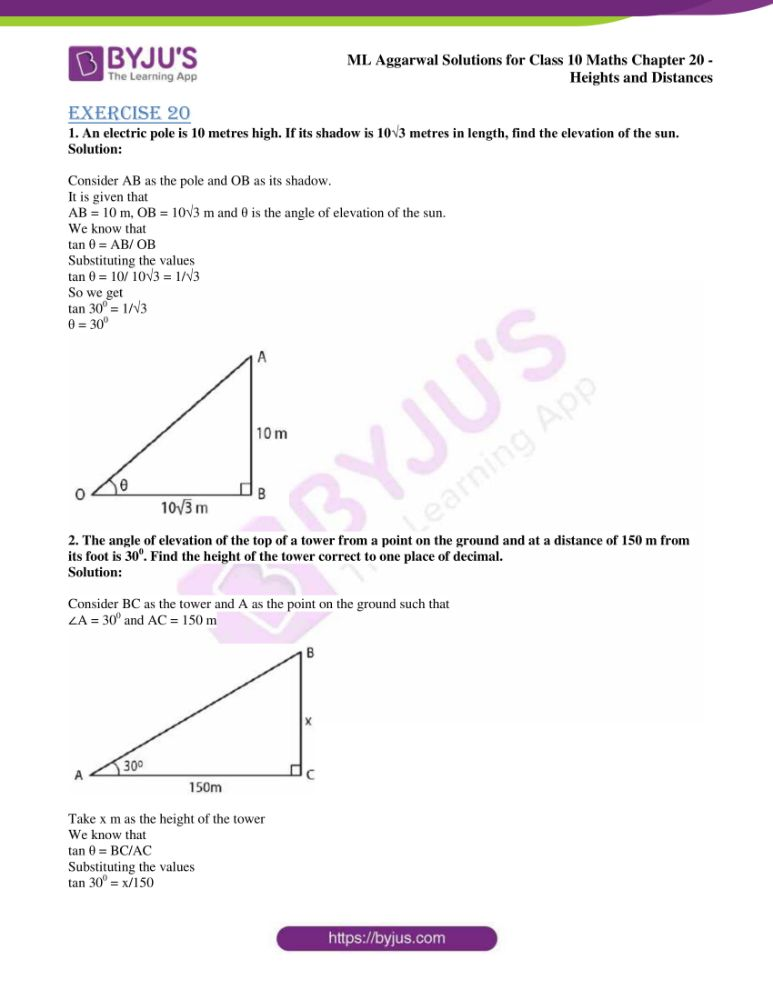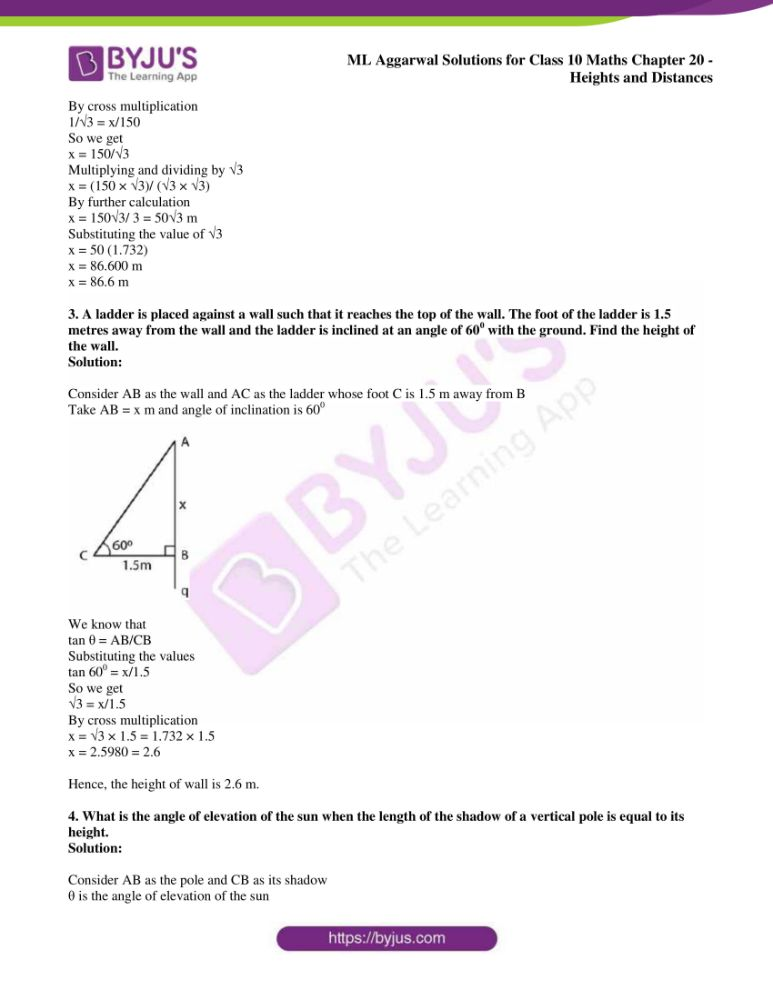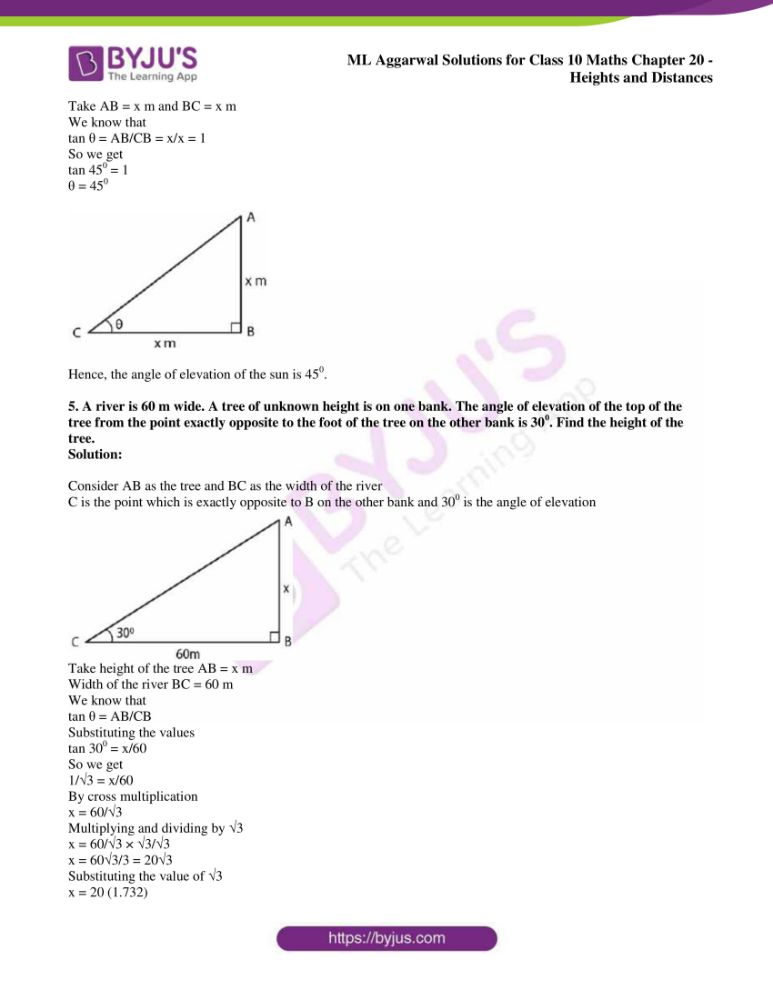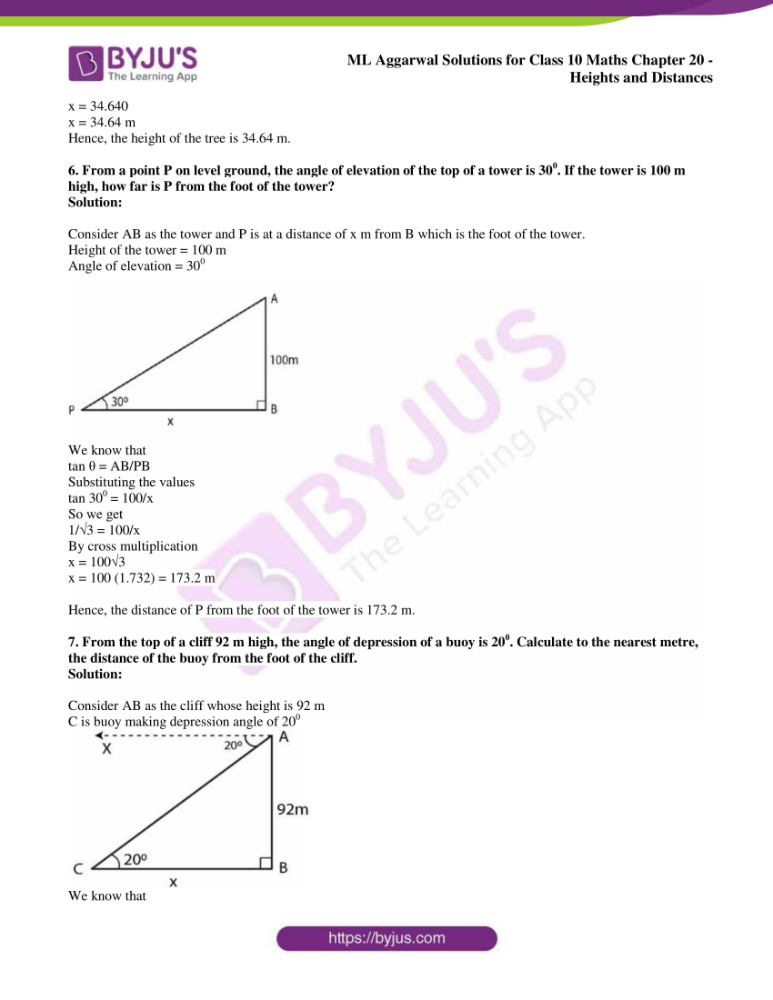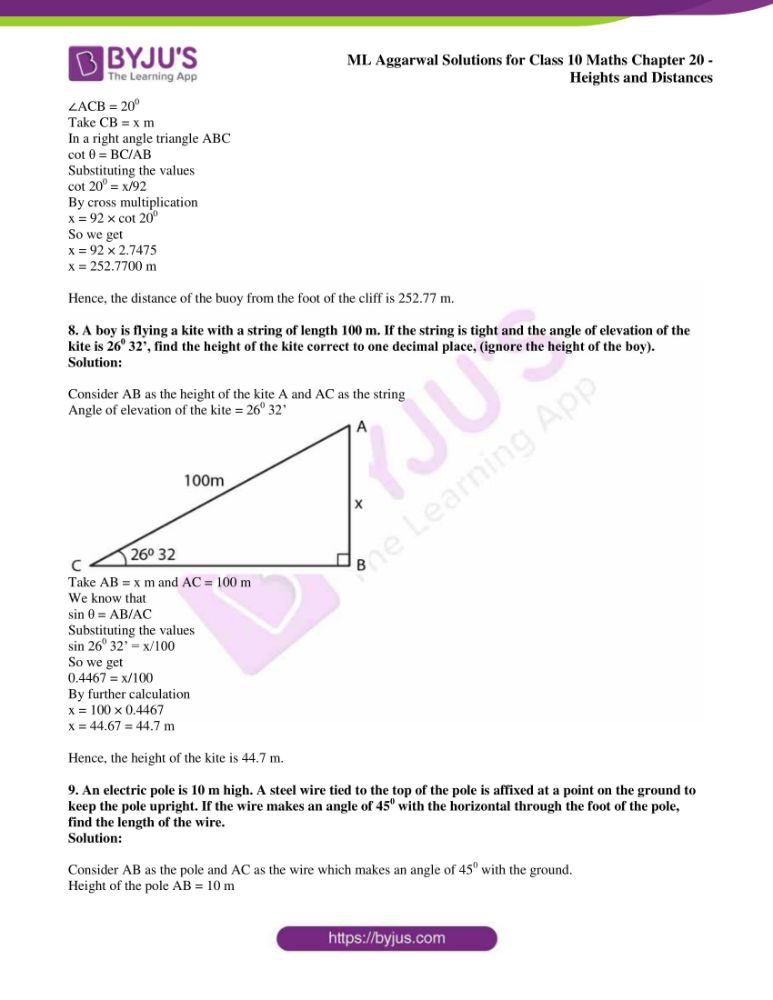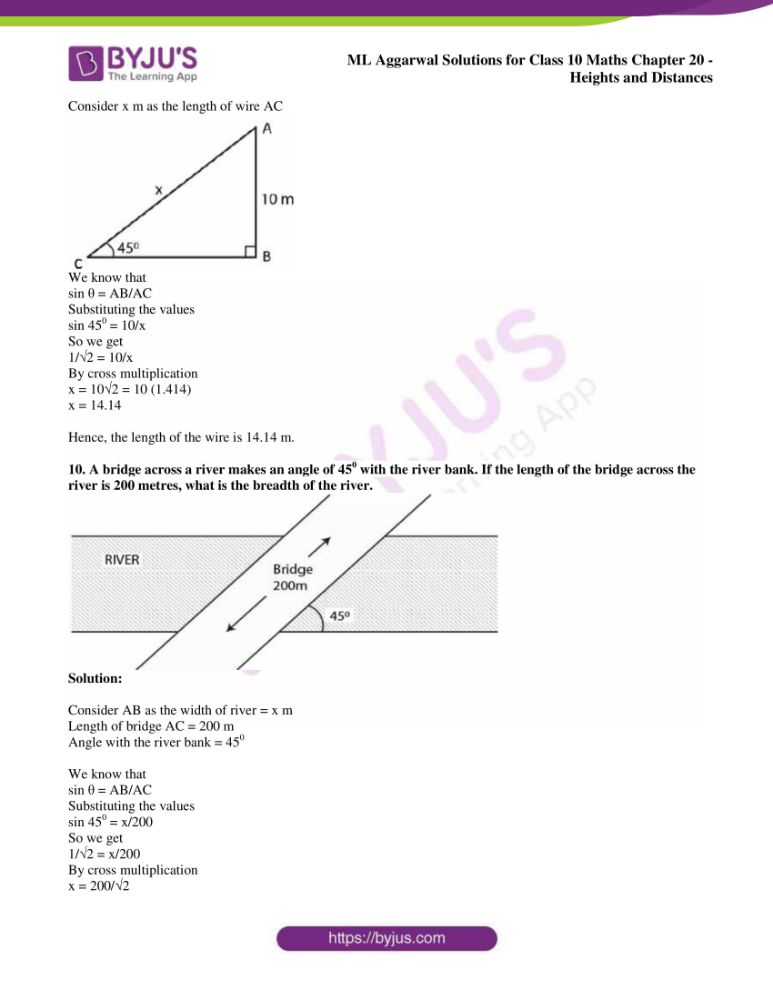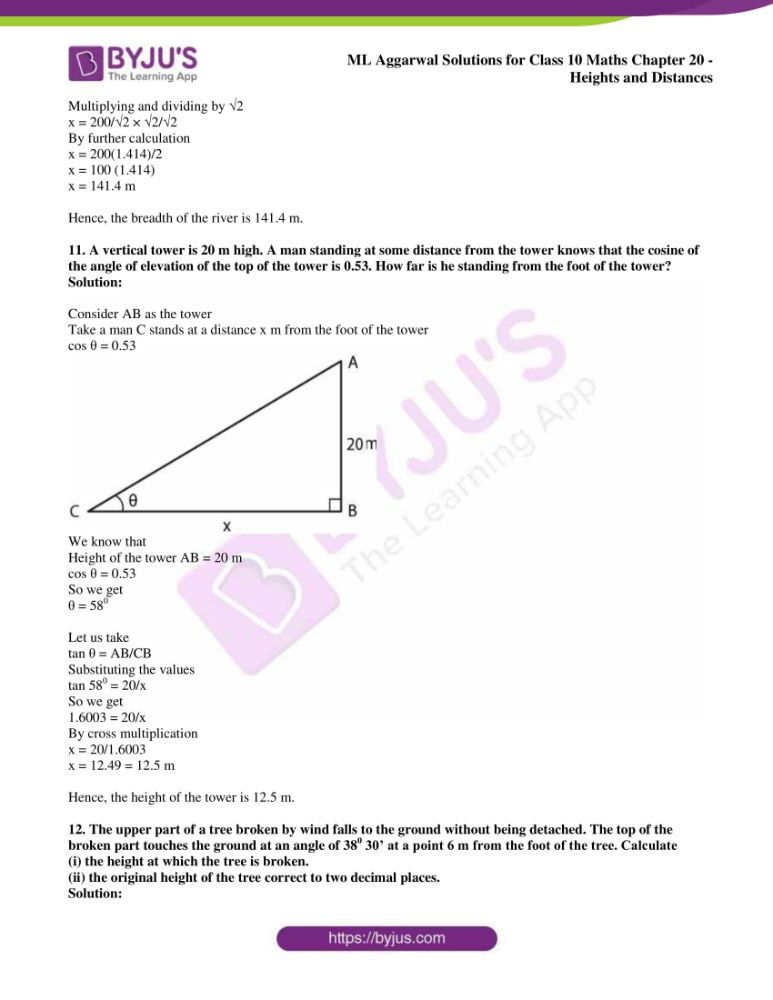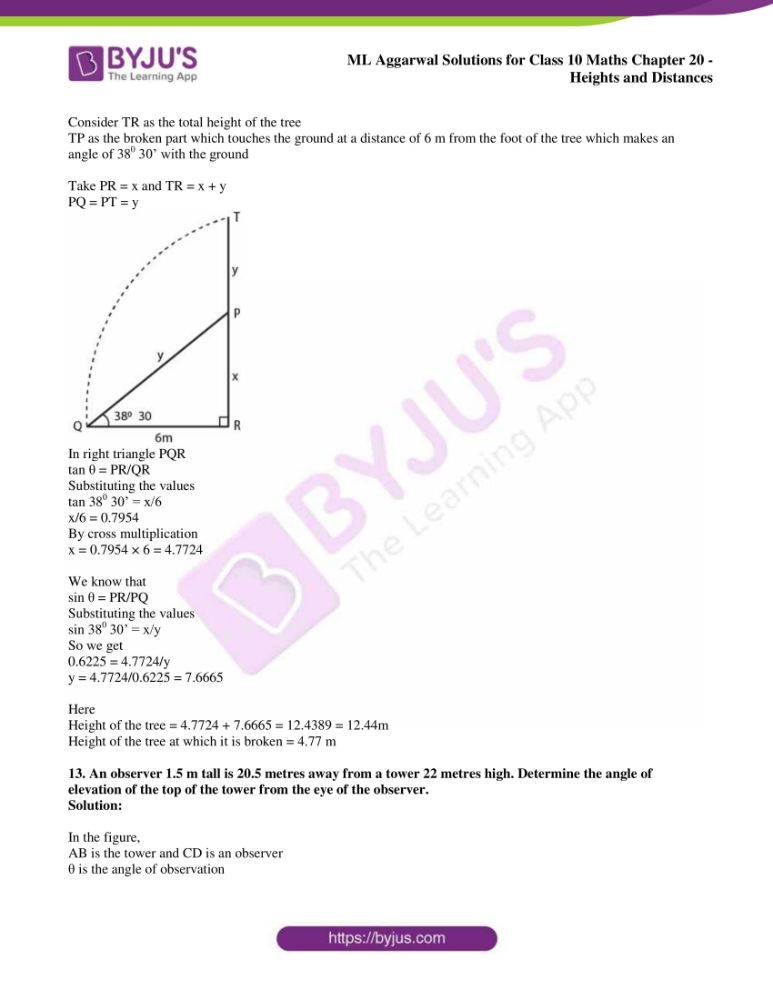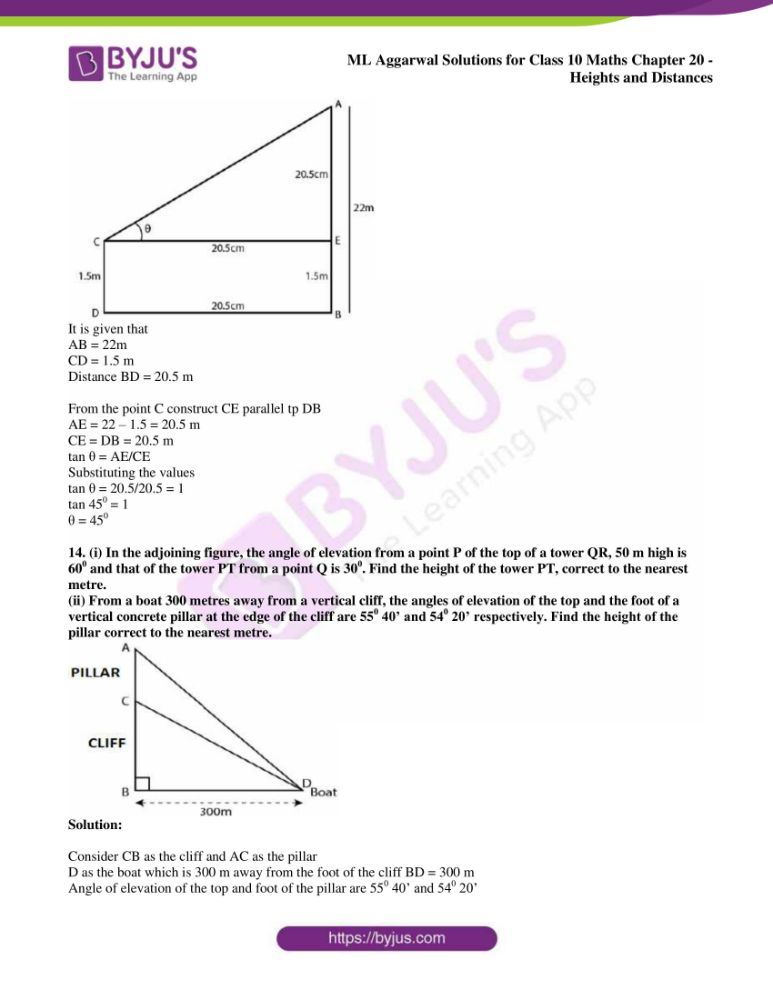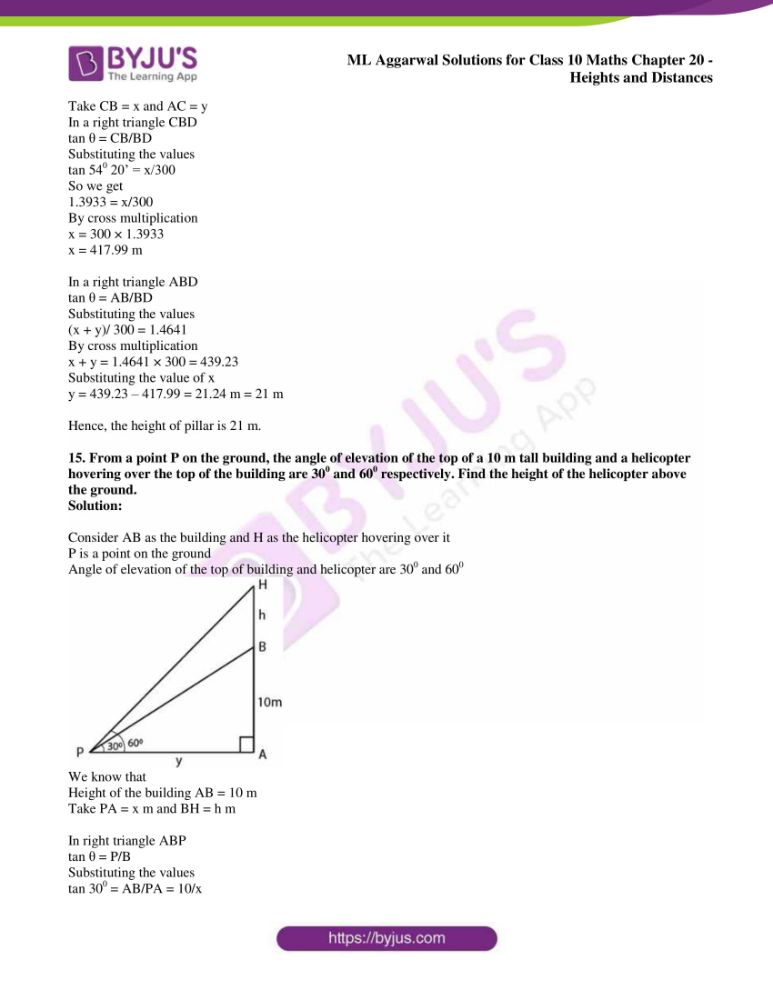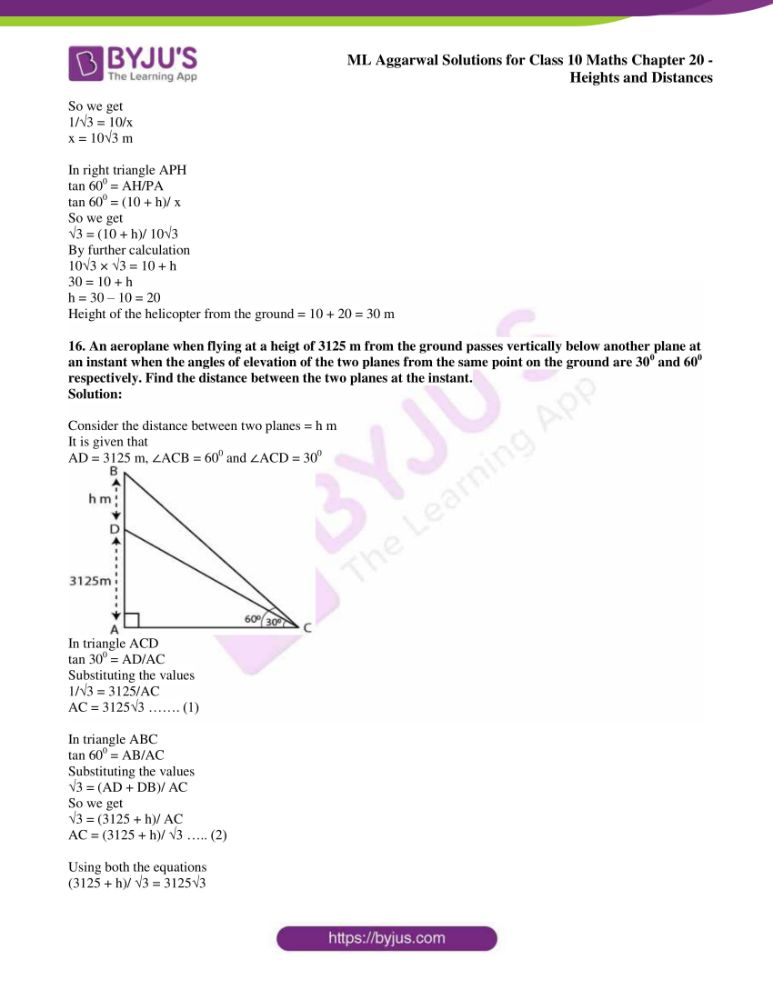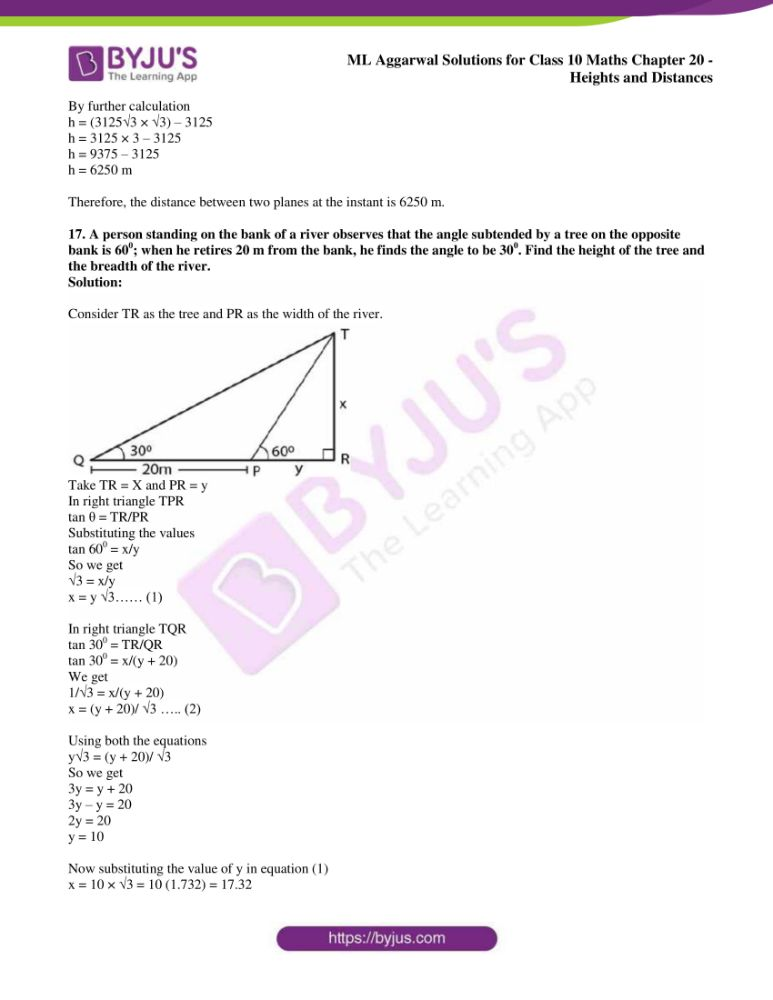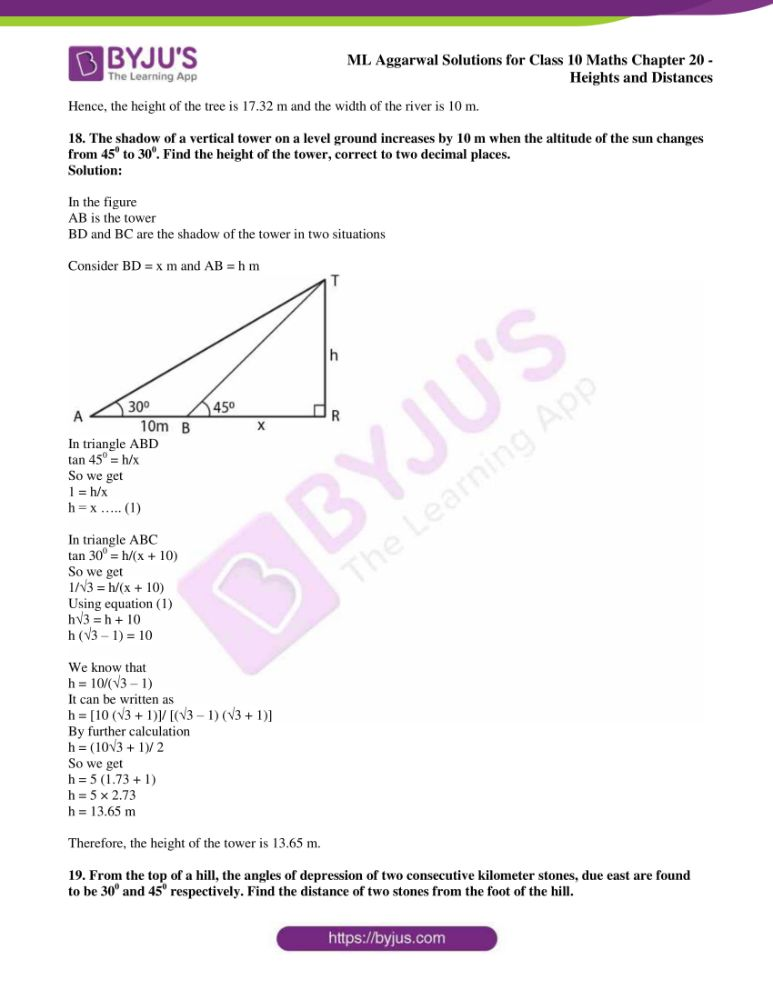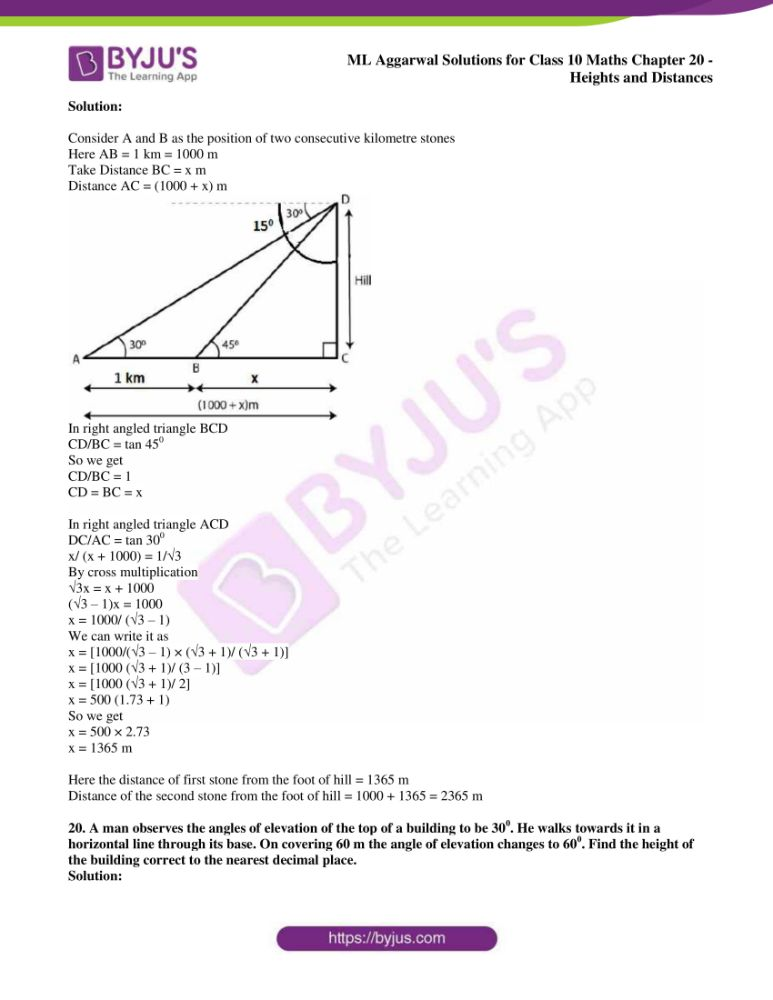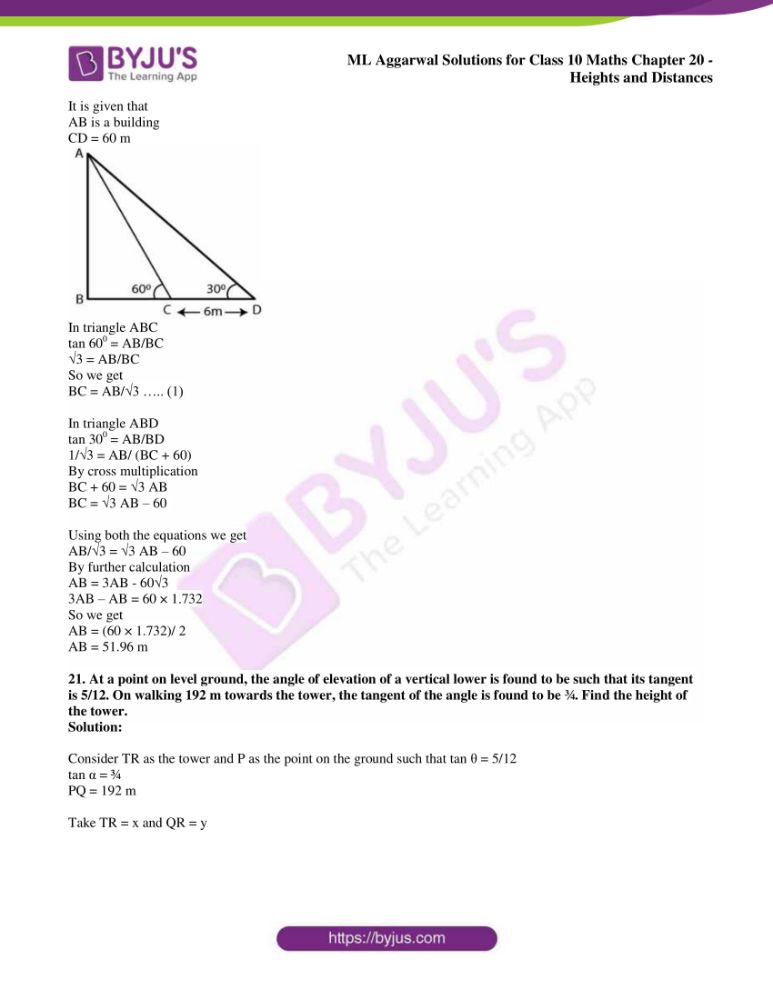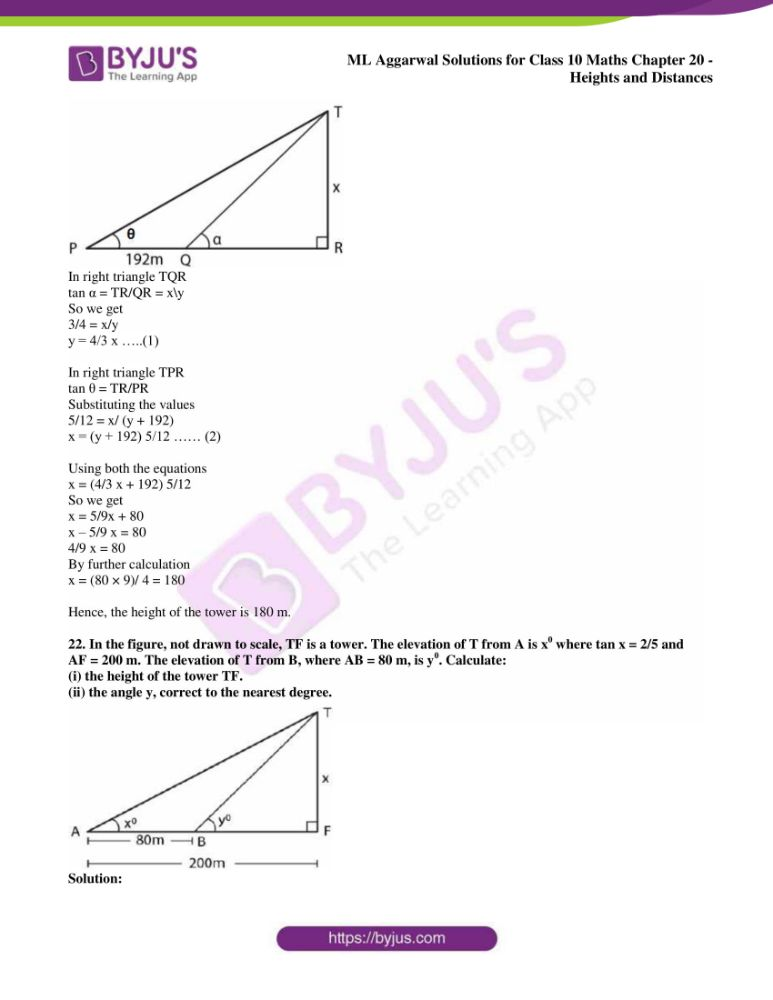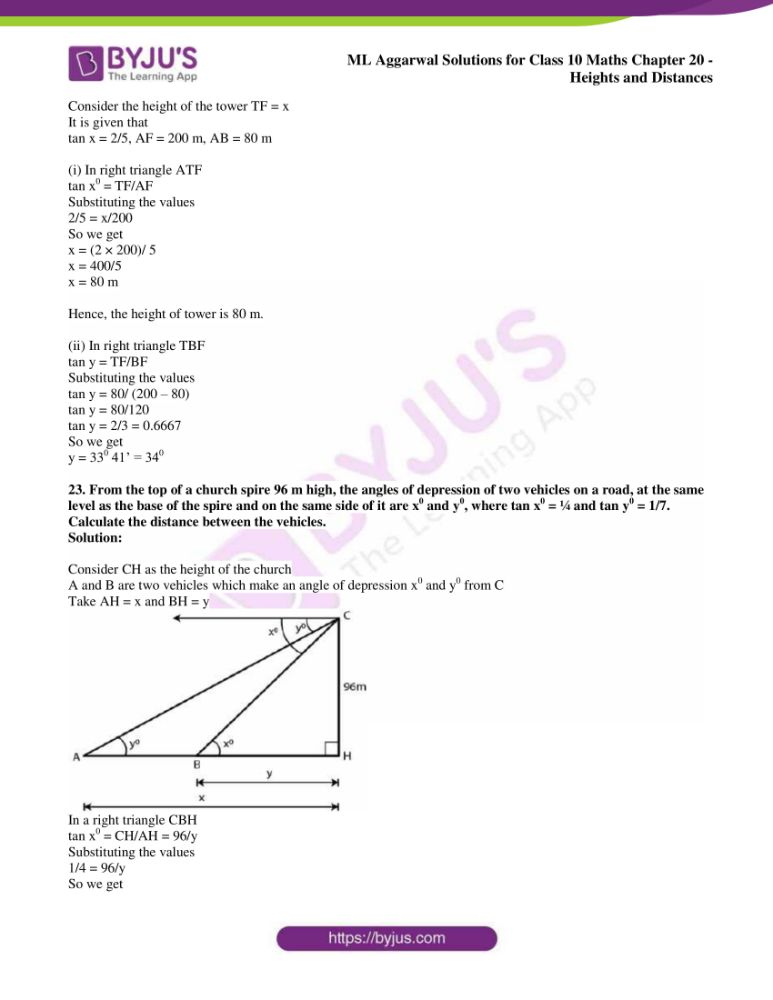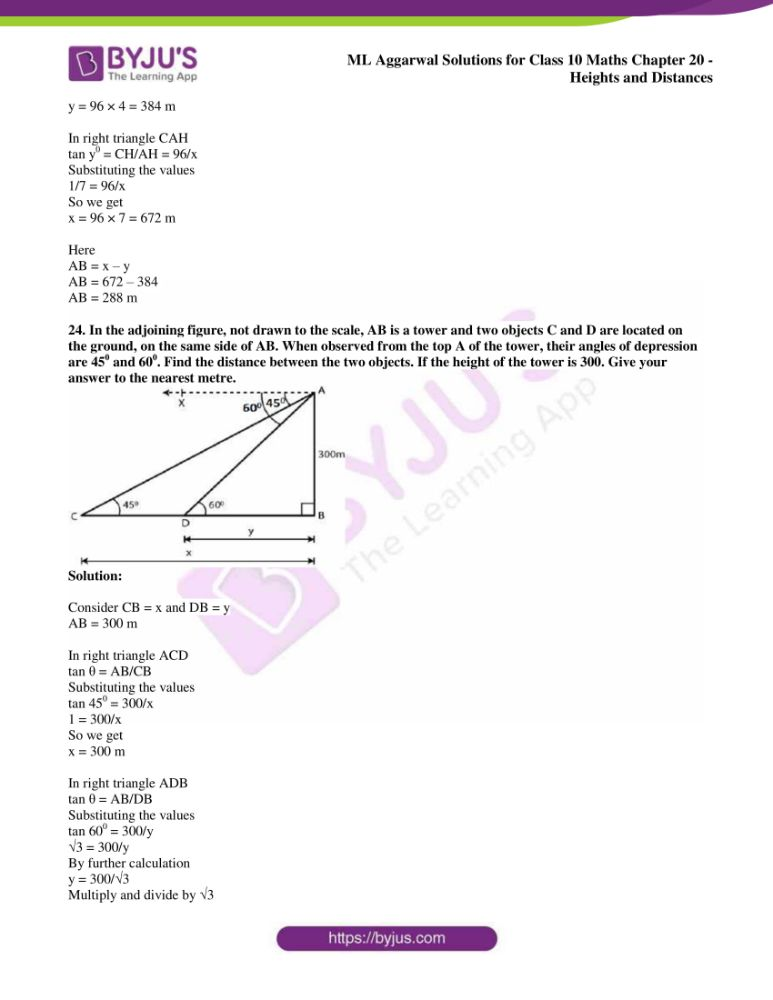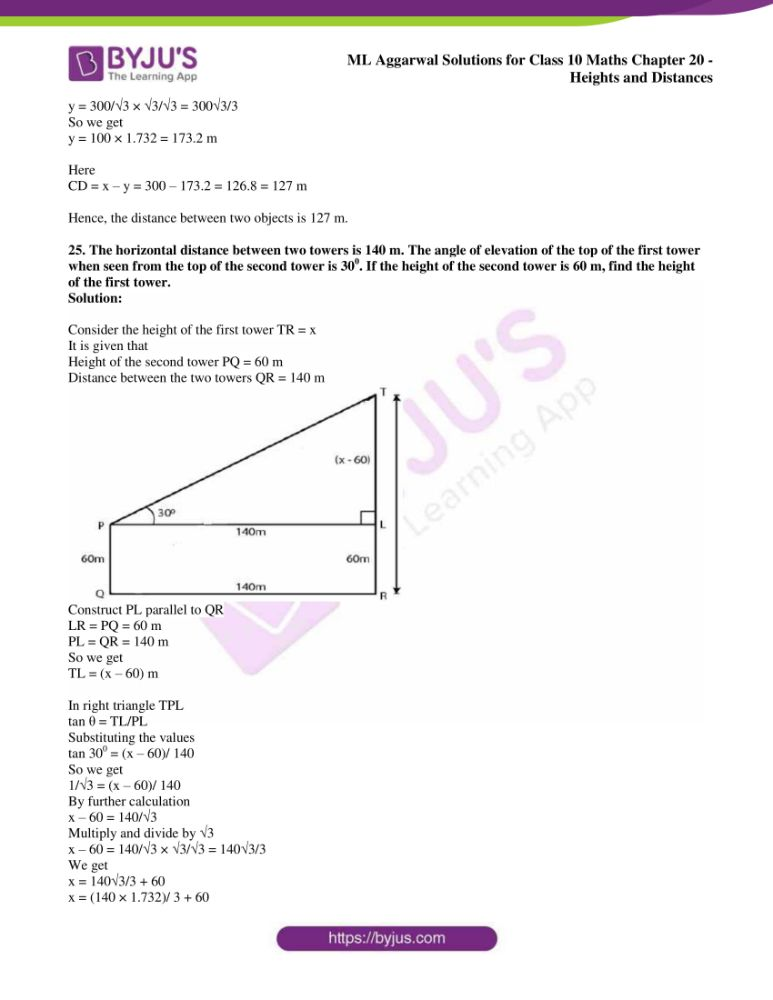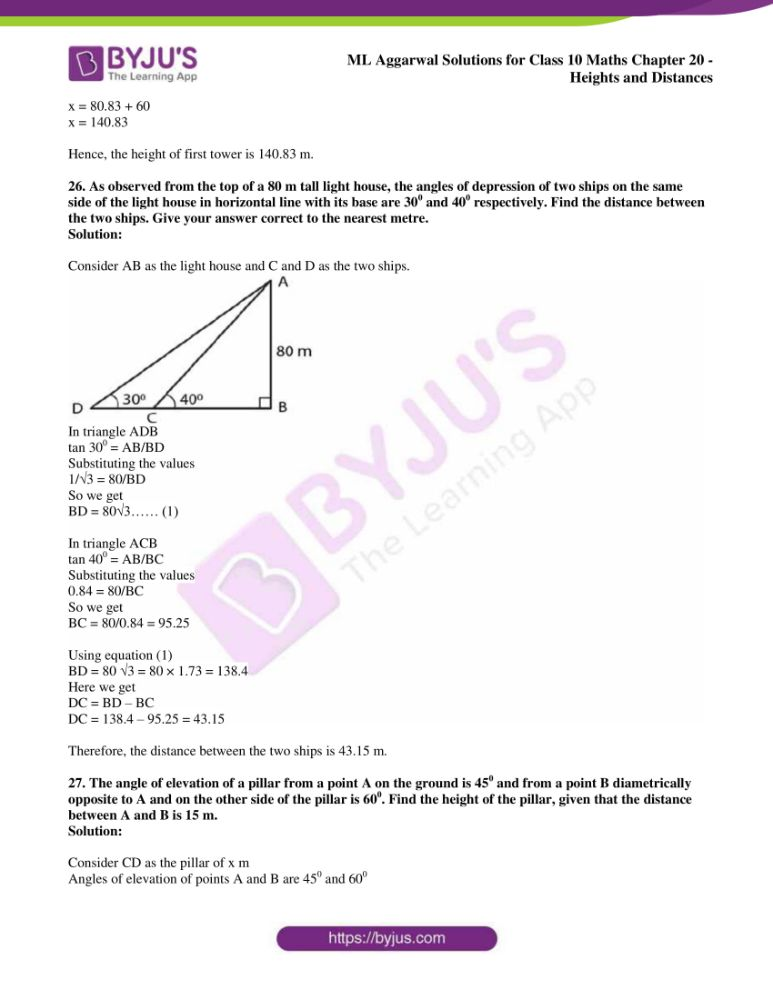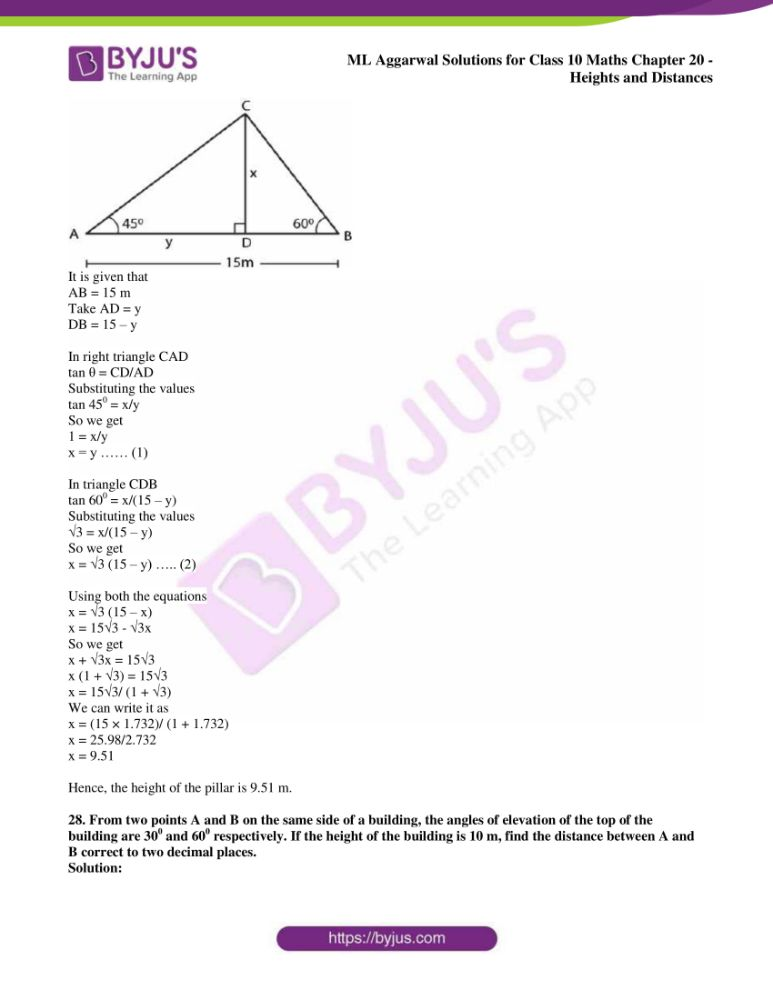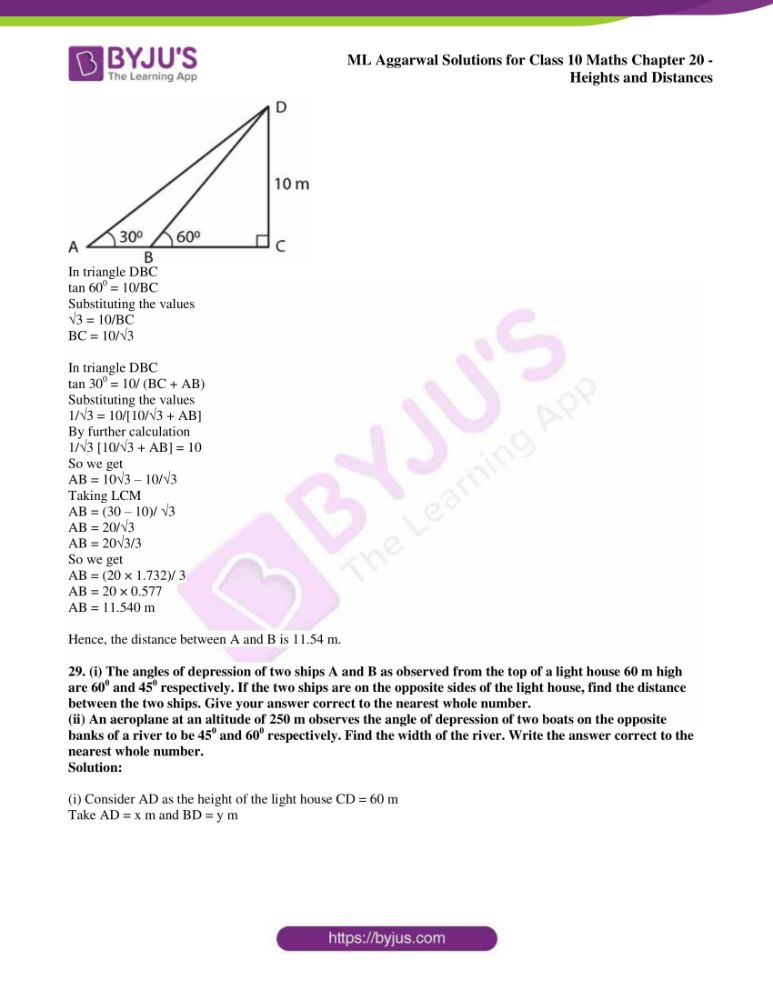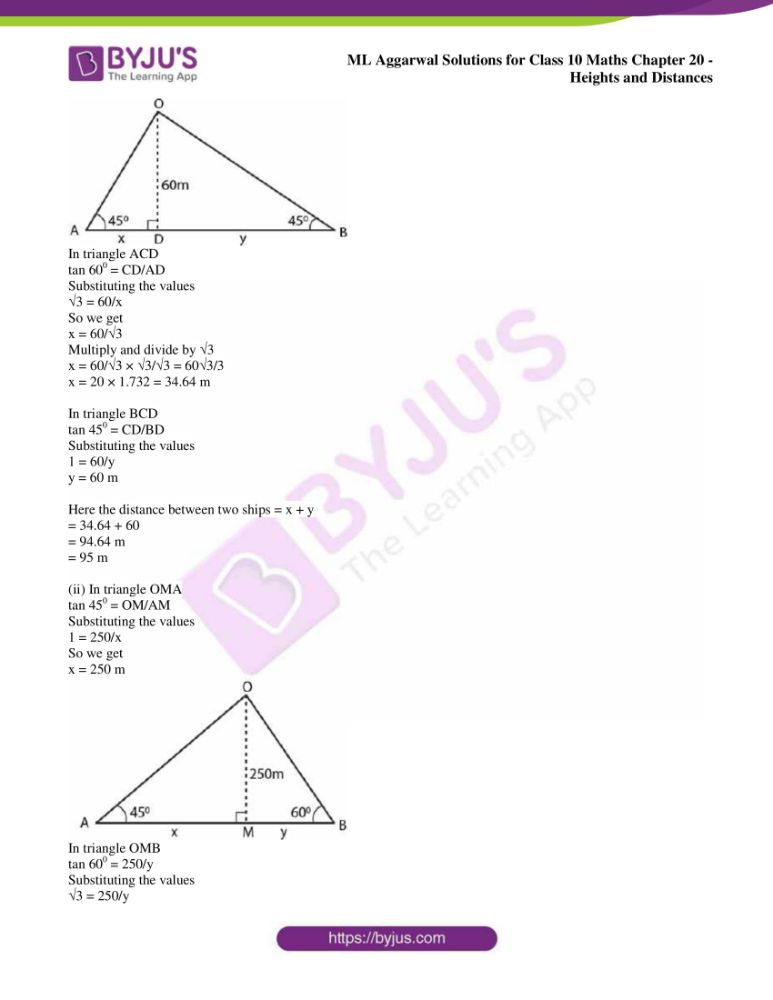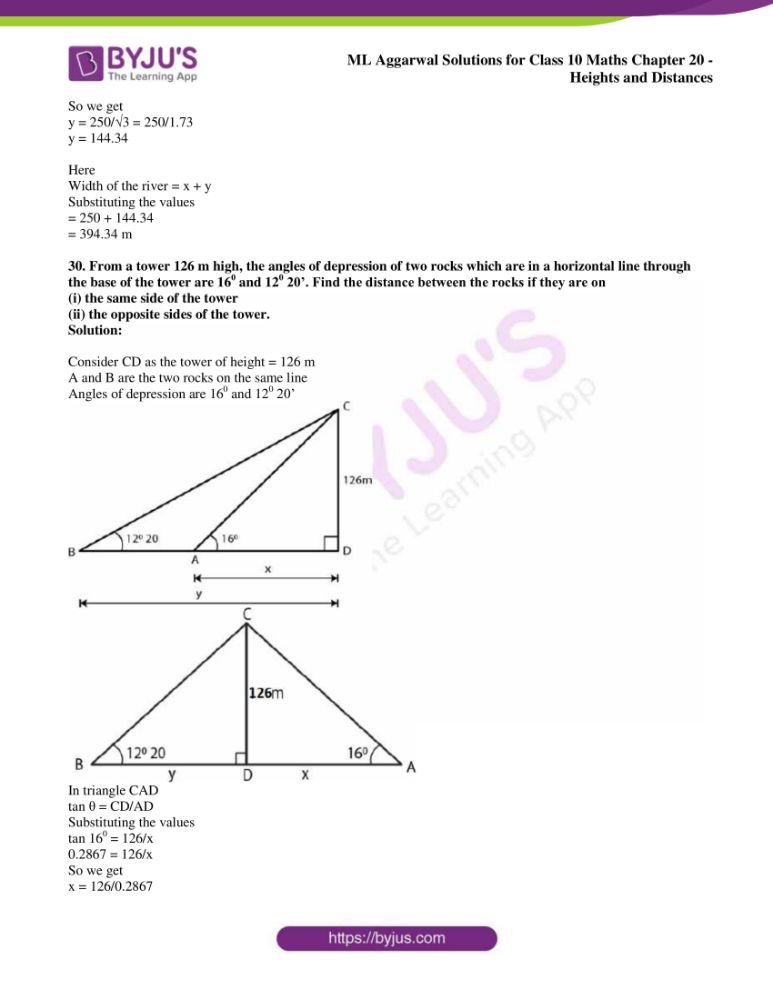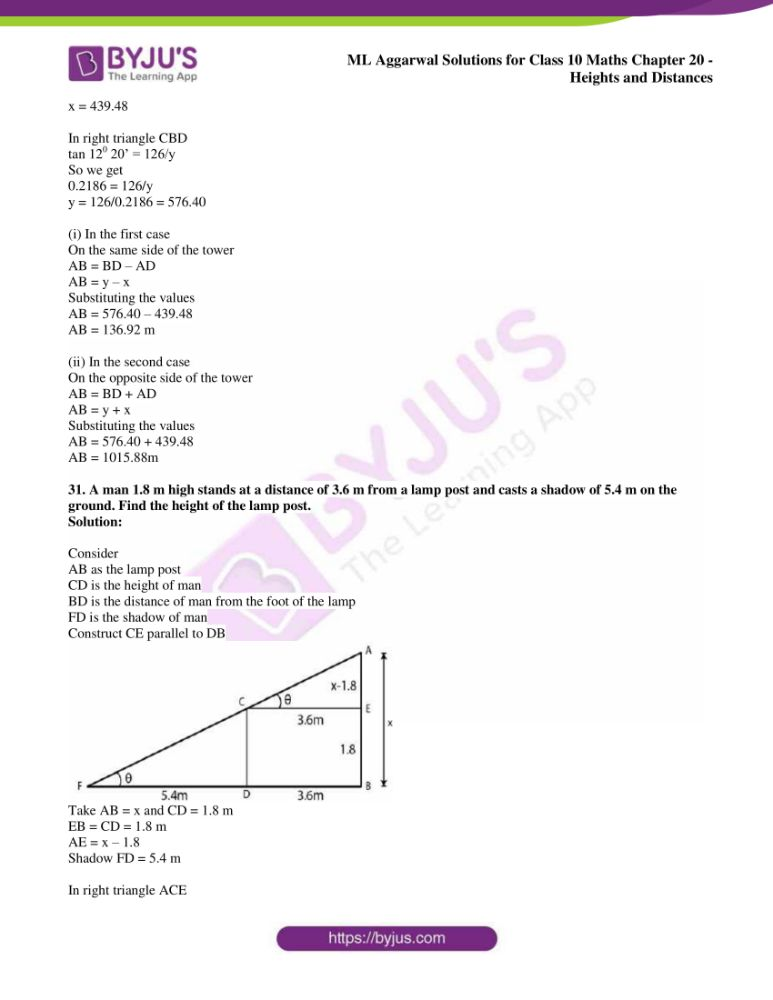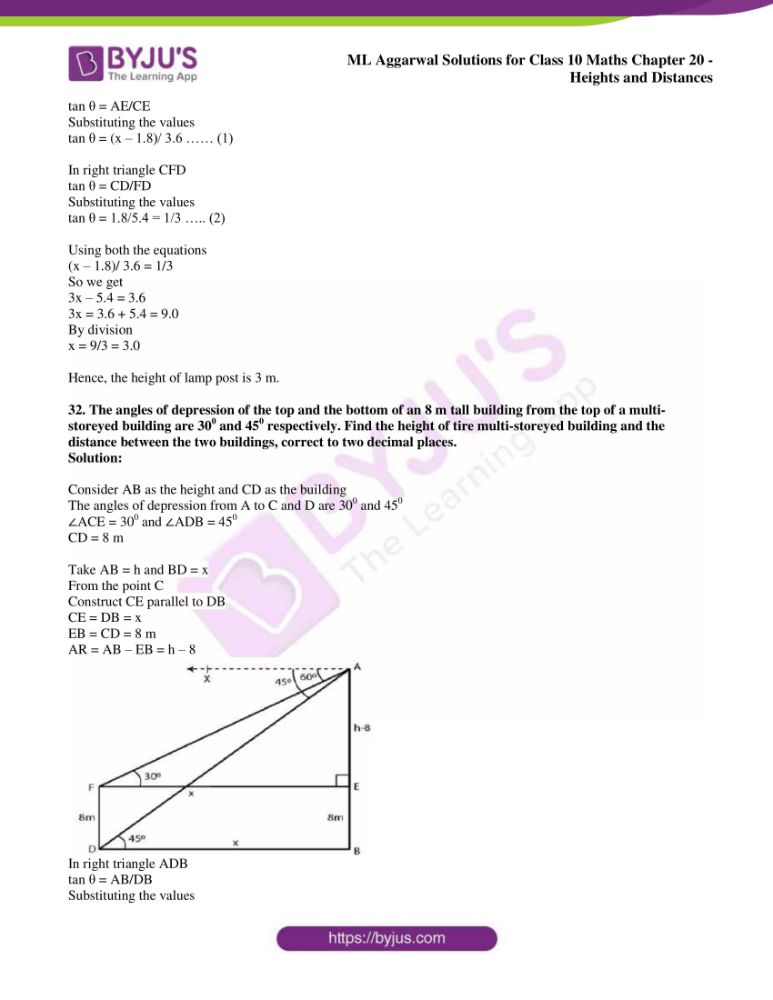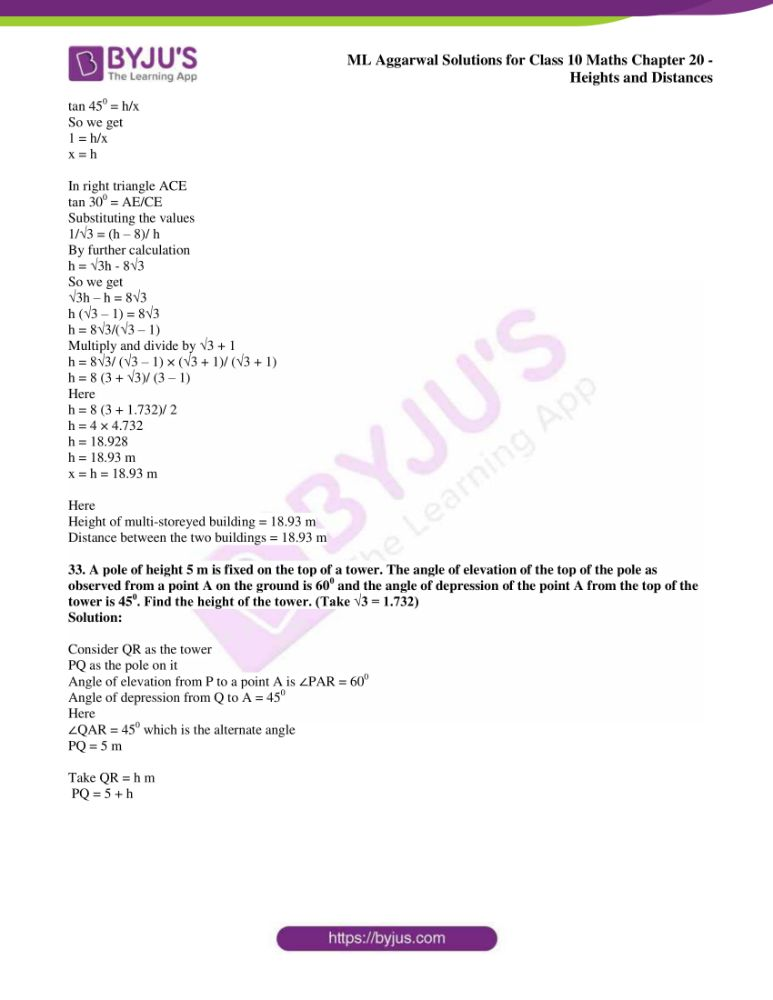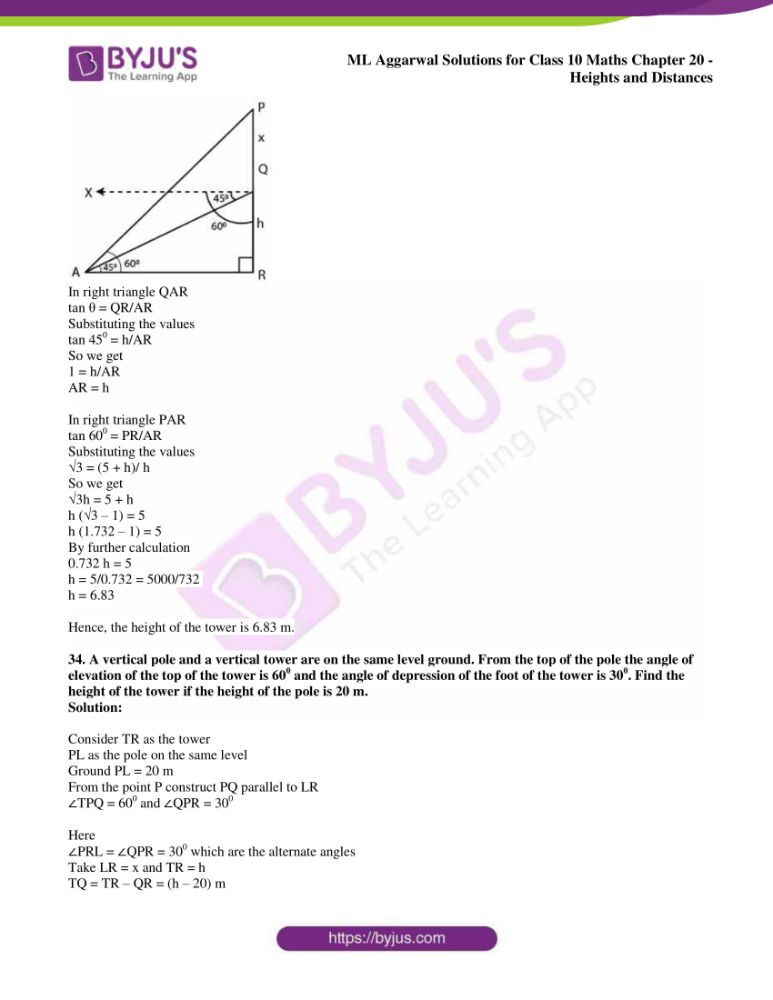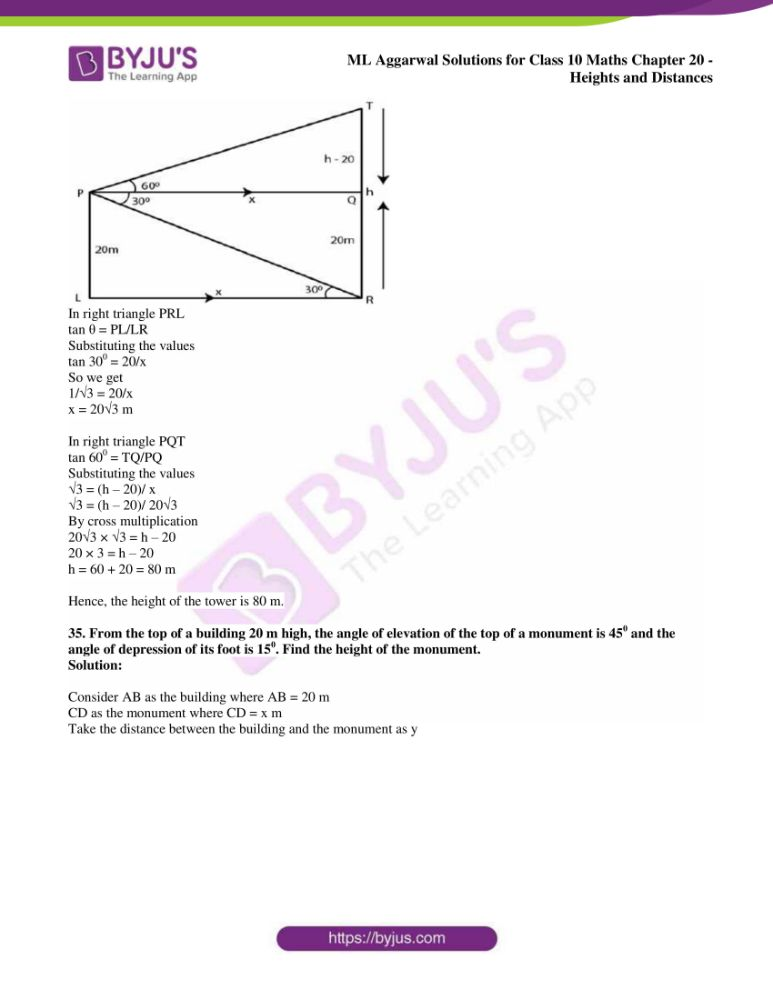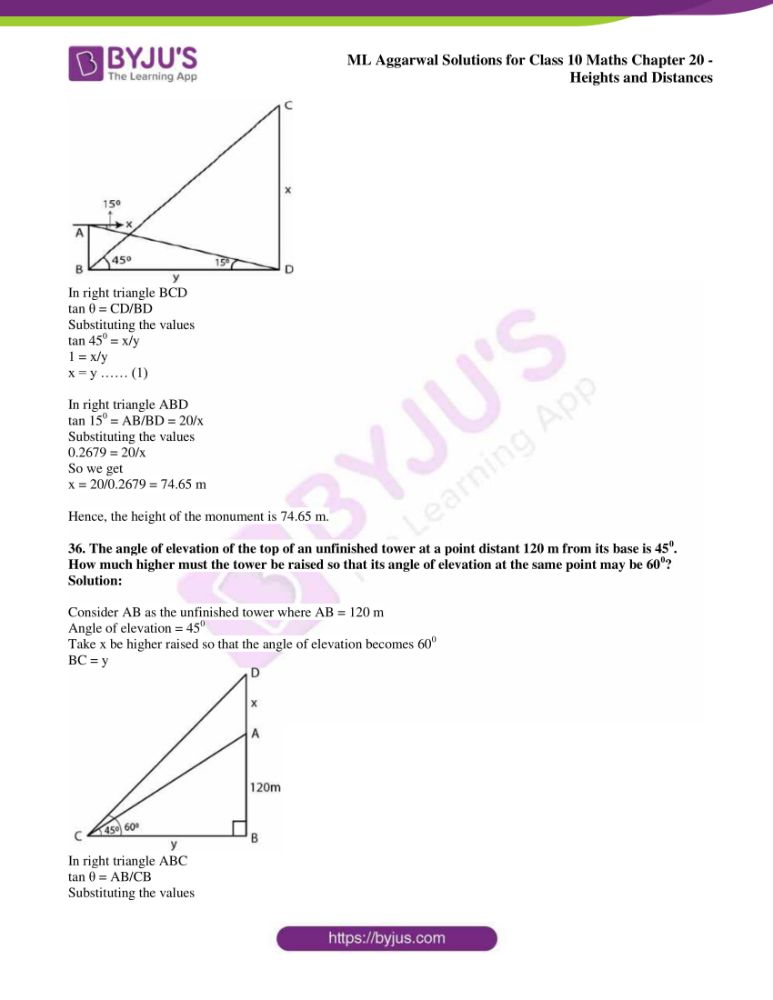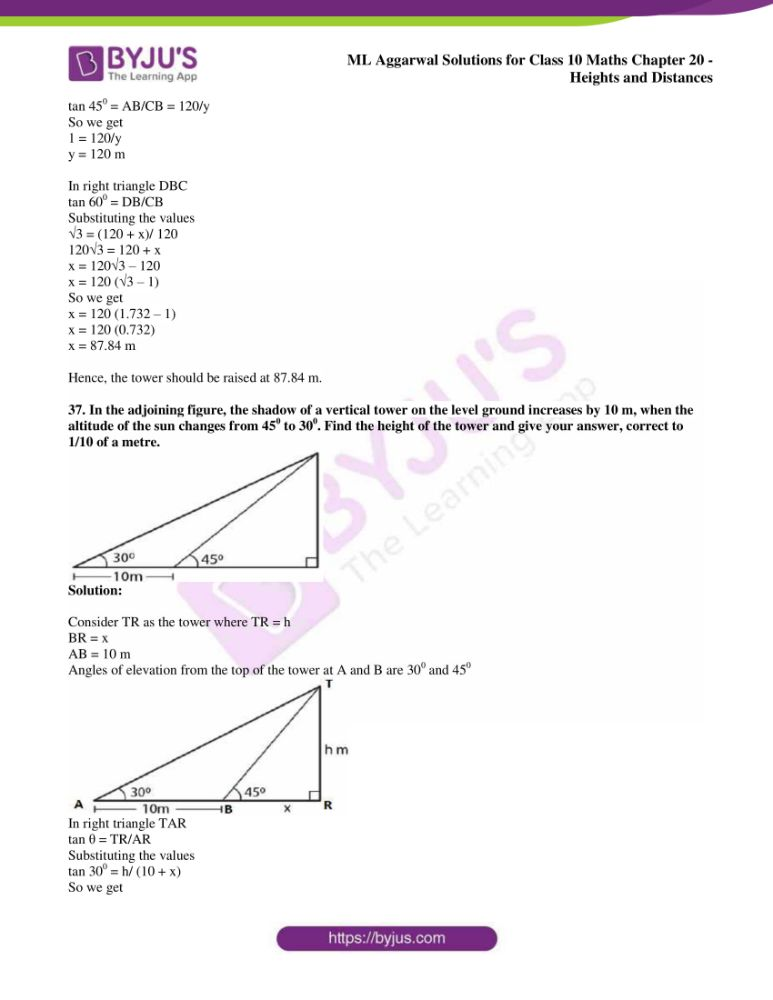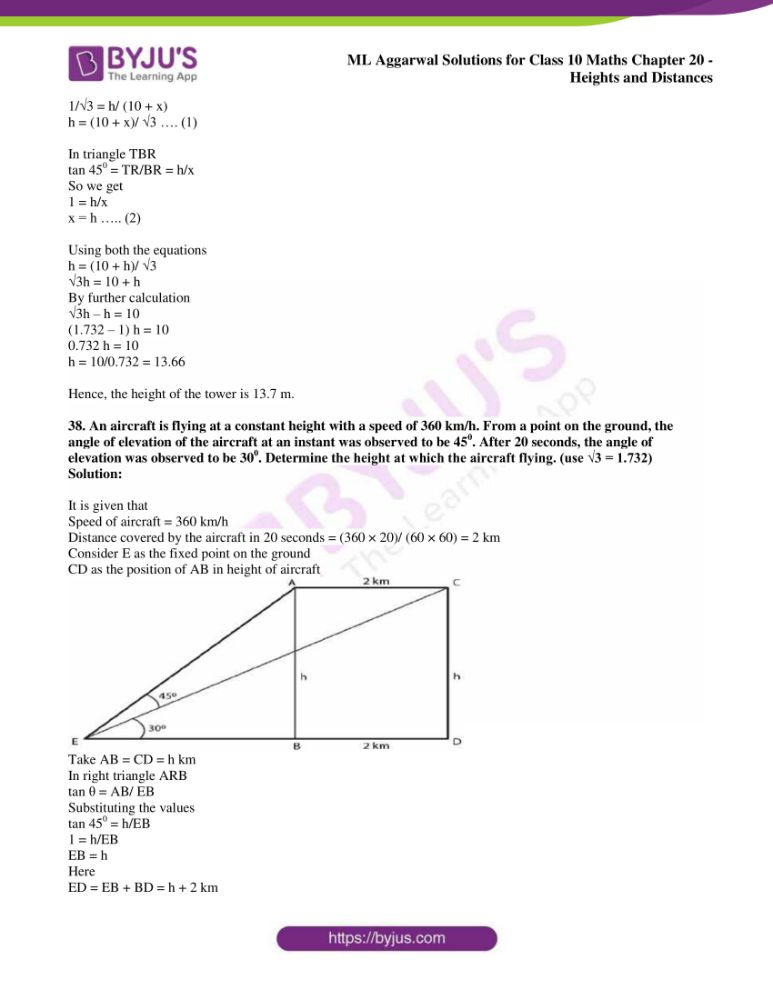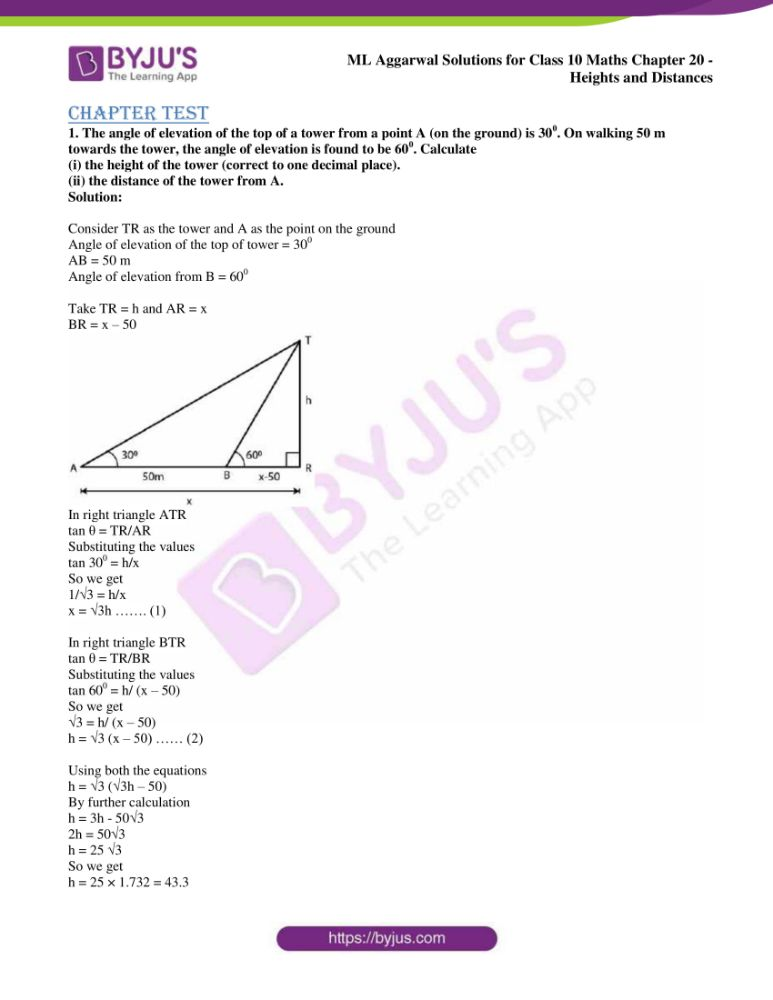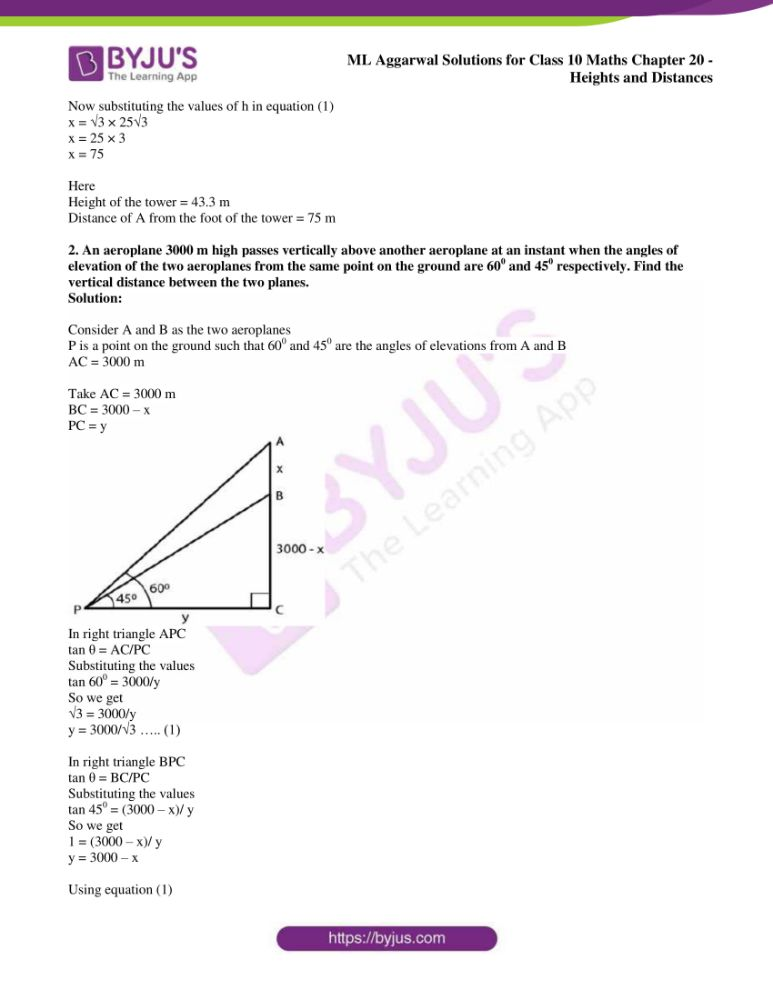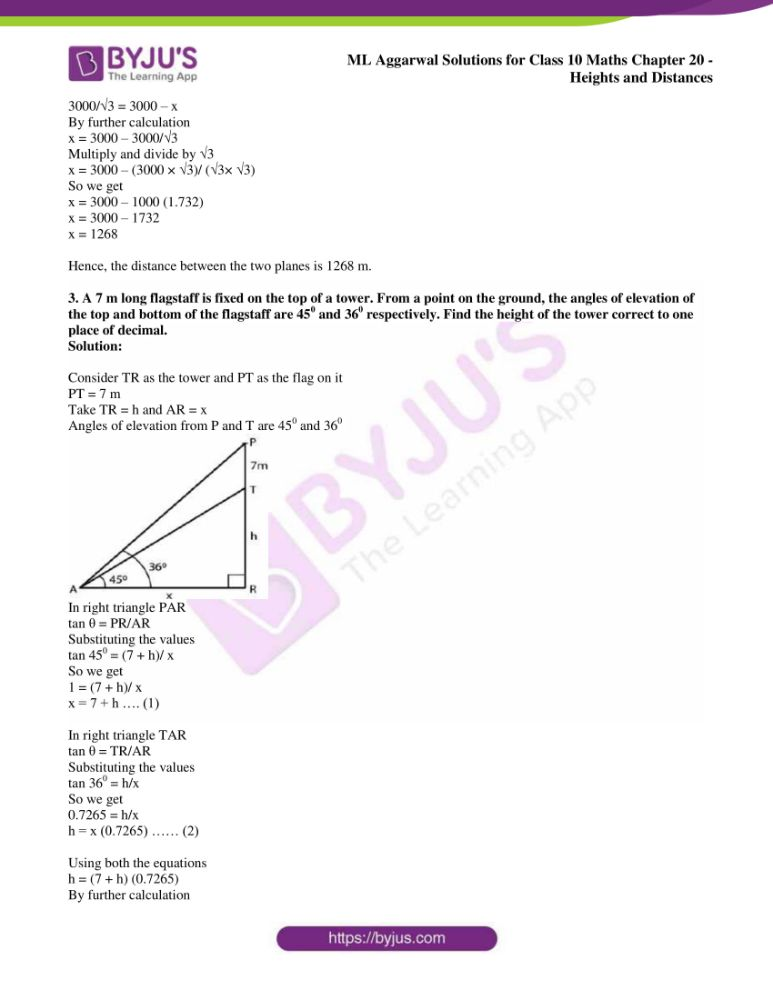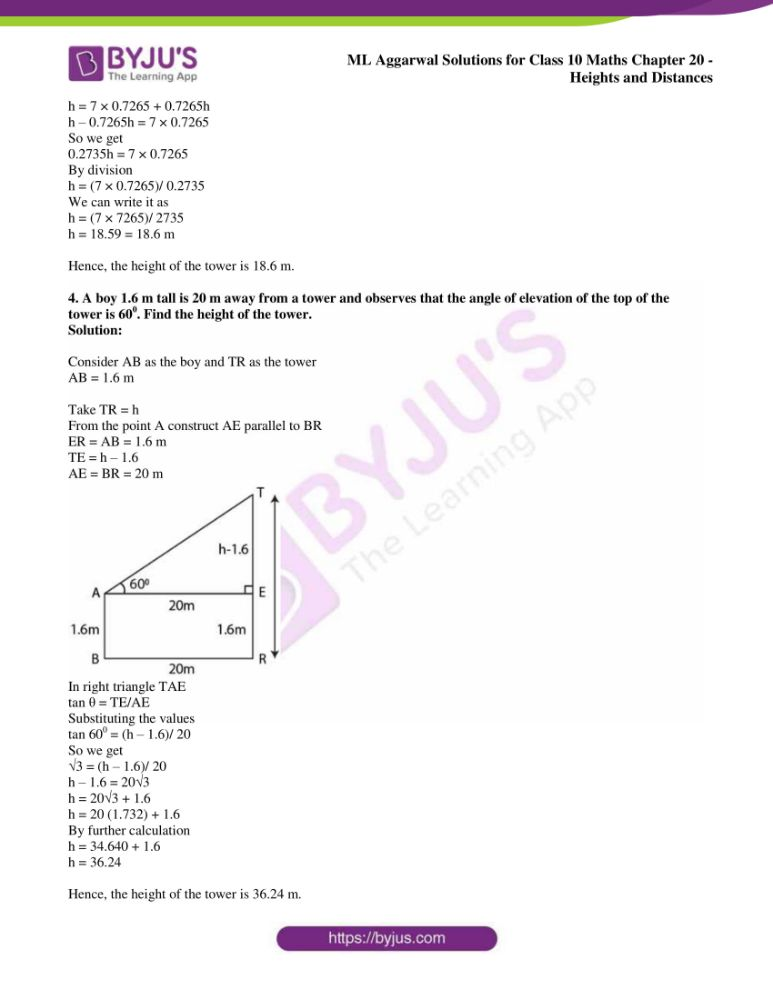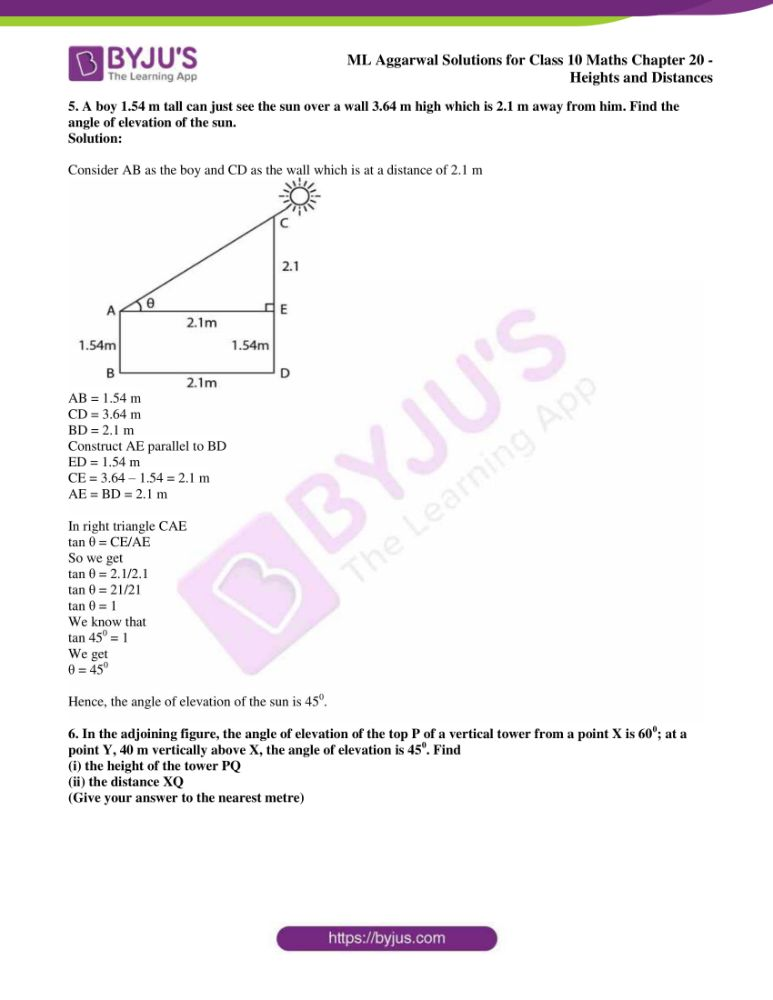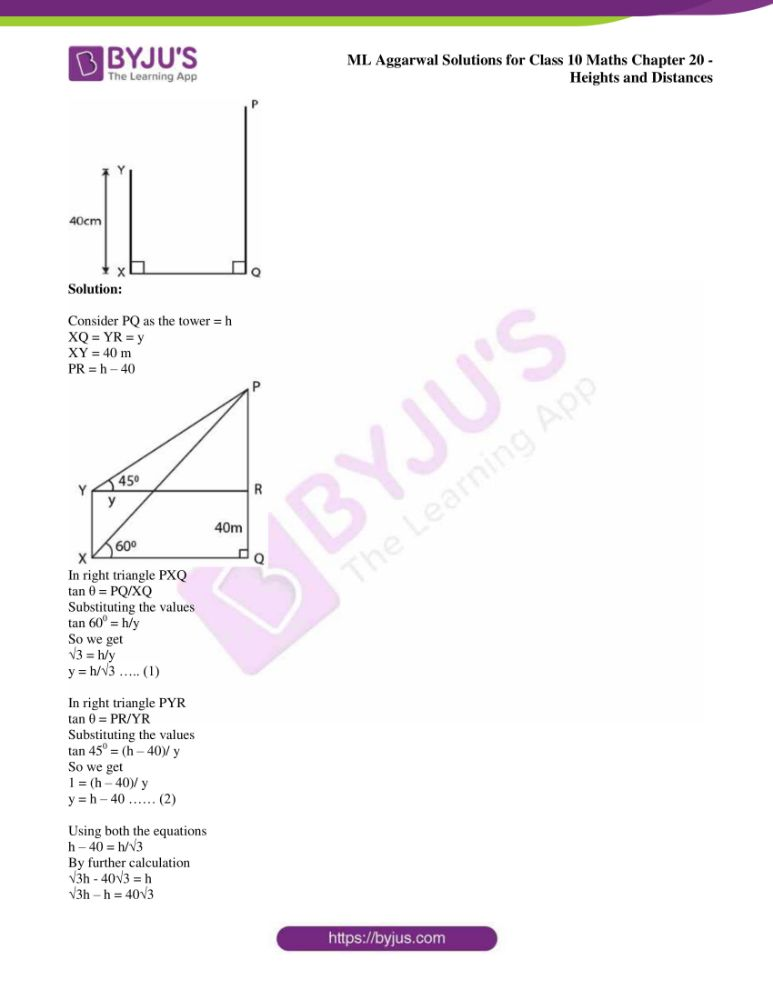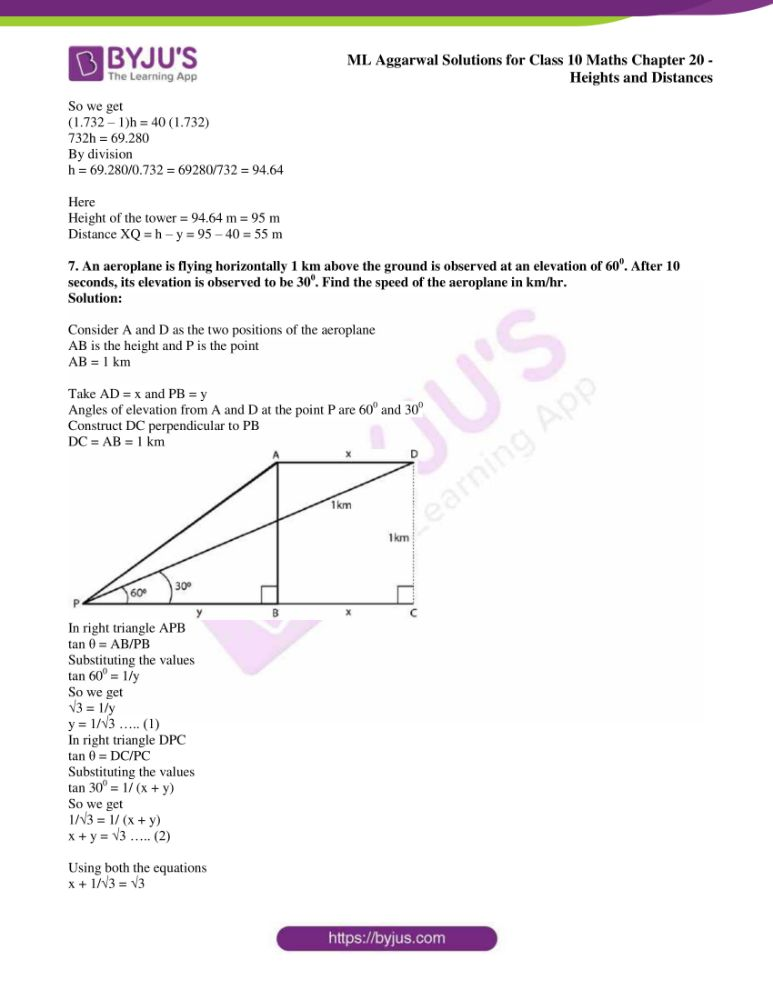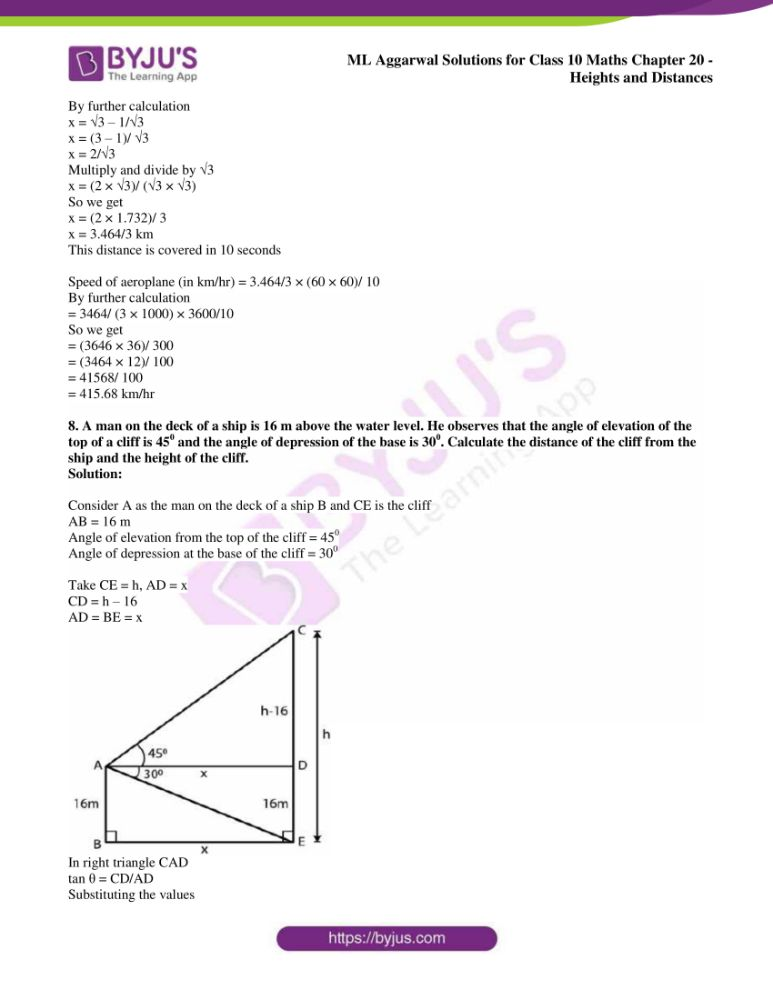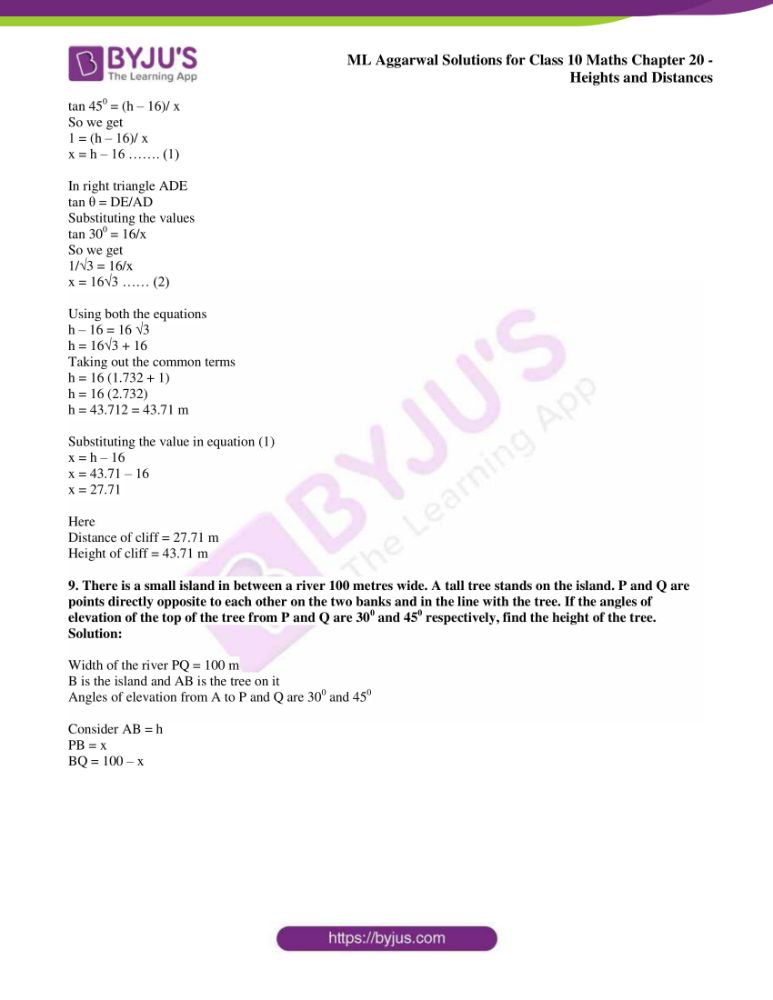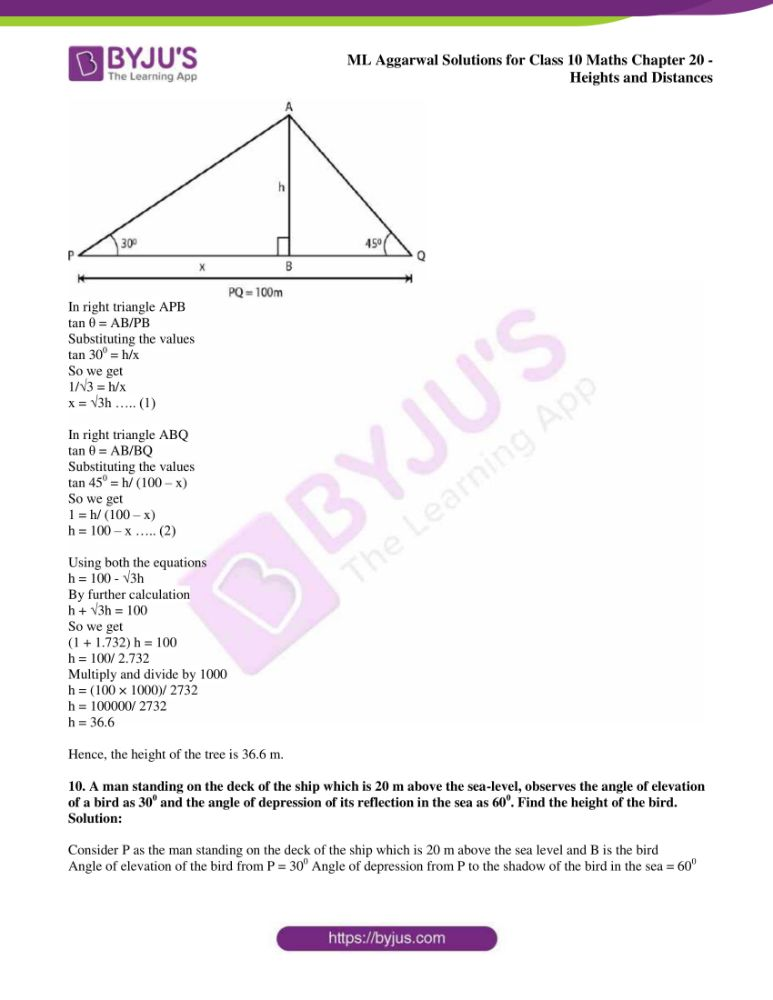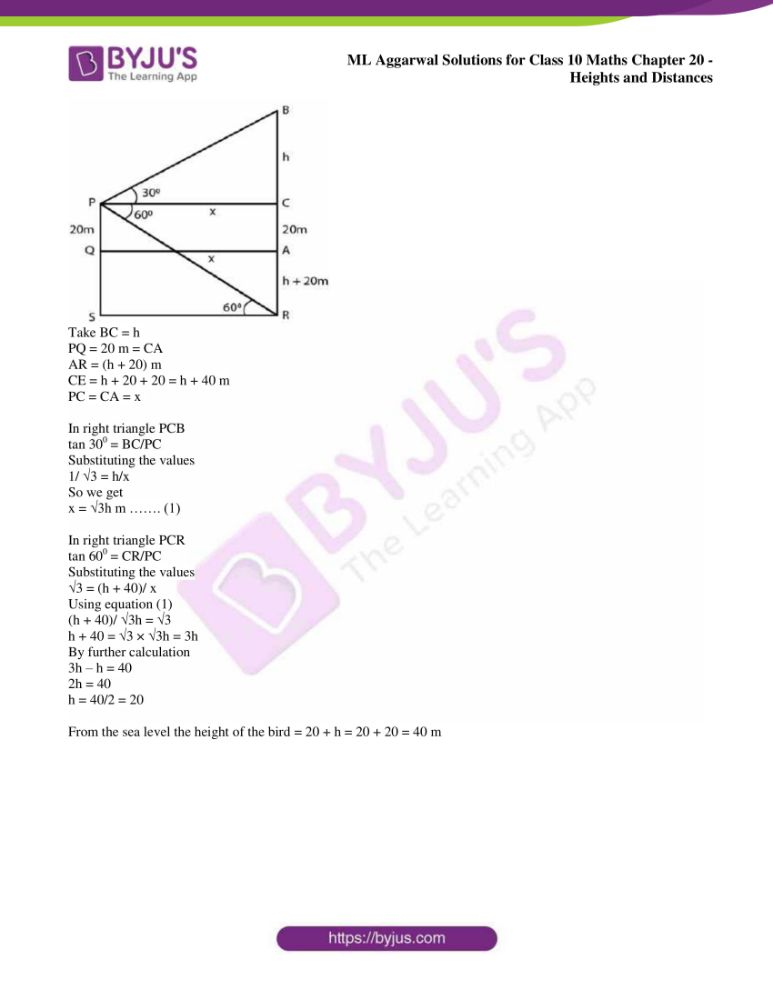## Access ML Aggarwal Solutions for Class 10 Maths Chapter 20: Heights and Distances

Exercise 20

1. An electric pole is 10 metres high. If its shadow is 10√3 metres in length, find the elevation of the sun.

Solution:

Consider AB as the pole and OB as its shadow.

It is given that

AB = 10 m, OB = 10√3 m and θ is the angle of elevation of the sun.

We know that

tan θ = AB/ OB

Substituting the values

tan θ = 10/ 10√3 = 1/√3

So we get

tan 300 = 1/√3

θ = 300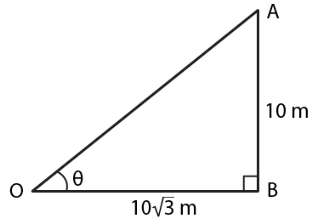2. The angle of elevation of the top of a tower from a point on the ground and at a distance of 150 m from its foot is 300. Find the height of the tower correct to one place of decimal.

Solution:

Consider BC as the tower and A as the point on the ground such that

∠A = 300 and AC = 150 m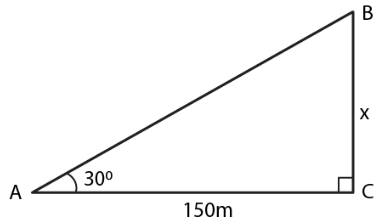Take x m as the height of the tower

We know that

tan θ = BC/AC

Substituting the values

tan 300 = x/150

By cross multiplication

1/√3 = x/150

So we get

x = 150/√3

Multiplying and dividing by √3

x = (150 × √3)/ (√3 × √3)

By further calculation

x = 150√3/ 3 = 50√3 m

Substituting the value of √3

x = 50 (1.732)

x = 86.600 m

x = 86.6 m

3. A ladder is placed against a wall such that it reaches the top of the wall. The foot of the ladder is 1.5 metres away from the wall and the ladder is inclined at an angle of 600 with the ground. Find the height of the wall.

Solution:

Consider AB as the wall and AC as the ladder whose foot C is 1.5 m away from B

Take AB = x m and angle of inclination is 600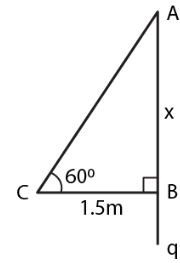We know that

tan θ = AB/CB

Substituting the values

tan 600 = x/1.5

So we get

√3 = x/1.5

By cross multiplication

x = √3 × 1.5 = 1.732 × 1.5

x = 2.5980 = 2.6

Hence, the height of wall is 2.6 m.

4. What is the angle of elevation of the sun when the length of the shadow of a vertical pole is equal to its height.

Solution:

Consider AB as the pole and CB as its shadow

θ is the angle of elevation of the sun

Take AB = x m and BC = x m

We know that

tan θ = AB/CB = x/x = 1

So we get

tan 450 = 1

θ = 450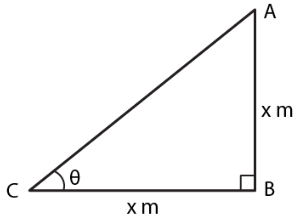Hence, the angle of elevation of the sun is 450.

5. A river is 60 m wide. A tree of unknown height is on one bank. The angle of elevation of the top of the tree from the point exactly opposite to the foot of the tree on the other bank is 300. Find the height of the tree.

Solution:

Consider AB as the tree and BC as the width of the river

C is the point which is exactly opposite to B on the other bank and 300 is the angle of elevation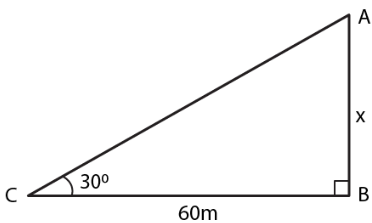Take height of the tree AB = x m

Width of the river BC = 60 m

We know that

tan θ = AB/CB

Substituting the values

tan 300 = x/60

So we get

1/√3 = x/60

By cross multiplication

x = 60/√3

Multiplying and dividing by √3

x = 60/√3 × √3/√3

x = 60√3/3 = 20√3

Substituting the value of √3

x = 20 (1.732)

x = 34.640

x = 34.64 m

Hence, the height of the tree is 34.64 m.

6. From a point P on level ground, the angle of elevation of the top of a tower is 300. If the tower is 100 m high, how far is P from the foot of the tower?

Solution:

Consider AB as the tower and P is at a distance of x m from B which is the foot of the tower.

Height of the tower = 100 m

Angle of elevation = 300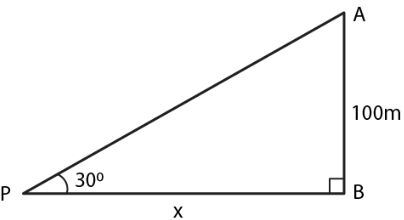We know that

tan θ = AB/PB

Substituting the values

tan 300 = 100/x

So we get

1/√3 = 100/x

By cross multiplication

x = 100√3

x = 100 (1.732) = 173.2 m

Hence, the distance of P from the foot of the tower is 173.2 m.

7. From the top of a cliff 92 m high, the angle of depression of a buoy is 200. Calculate to the nearest metre, the distance of the buoy from the foot of the cliff.

Solution:

Consider AB as the cliff whose height is 92 m

C is buoy making depression angle of 200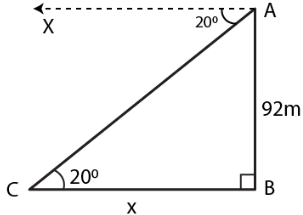We know that

∠ACB = 200

Take CB = x m

In a right angle triangle ABC

cot θ = BC/AB

Substituting the values

cot 200 = x/92

By cross multiplication

x = 92 × cot 200

So we get

x = 92 × 2.7475

x = 252.7700 m

Hence, the distance of the buoy from the foot of the cliff is 252.77 m.

8. A boy is flying a kite with a string of length 100 m. If the string is tight and the angle of elevation of the kite is 260 32’, find the height of the kite correct to one decimal place, (ignore the height of the boy).

Solution:

Consider AB as the height of the kite A and AC as the string

Angle of elevation of the kite = 260 32’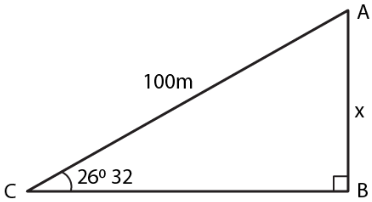Take AB = x m and AC = 100 m

We know that

sin θ = AB/AC

Substituting the values

sin 260 32’ = x/100

So we get

0.4467 = x/100

By further calculation

x = 100 × 0.4467

x = 44.67 = 44.7 m

Hence, the height of the kite is 44.7 m.

9. An electric pole is 10 m high. A steel wire tied to the top of the pole is affixed at a point on the ground to keep the pole upright. If the wire makes an angle of 450 with the horizontal through the foot of the pole, find the length of the wire.

Solution:

Consider AB as the pole and AC as the wire which makes an angle of 450 with the ground.

Height of the pole AB = 10 m

Consider x m as the length of wire AC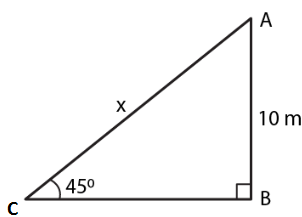We know that

sin θ = AB/AC

Substituting the values

sin 450 = 10/x

So we get

1/√2 = 10/x

By cross multiplication

x = 10√2 = 10 (1.414)

x = 14.14

Hence, the length of the wire is 14.14 m.

10. A bridge across a river makes an angle of 450 with the river bank. If the length of the bridge across the river is 200 metres, what is the breadth of the river.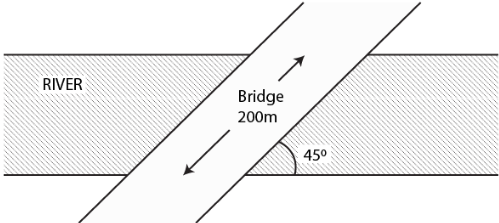Solution:

Consider AB as the width of river = x m

Length of bridge AC = 200 m

Angle with the river bank = 450

We know that

sin θ = AB/AC

Substituting the values

sin 450 = x/200

So we get

1/√2 = x/200

By cross multiplication

x = 200/√2

Multiplying and dividing by √2

x = 200/√2 × √2/√2

By further calculation

x = 200(1.414)/2

x = 100 (1.414)

x = 141.4 m

Hence, the breadth of the river is 141.4 m.

11. A vertical tower is 20 m high. A man standing at some distance from the tower knows that the cosine of the angle of elevation of the top of the tower is 0.53. How far is he standing from the foot of the tower?

Solution:

Consider AB as the tower

Take a man C stands at a distance x m from the foot of the tower

cos θ = 0.53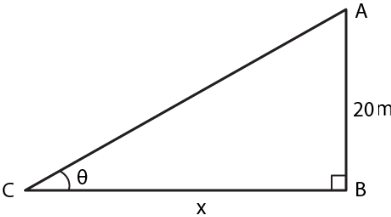We know that

Height of the tower AB = 20 m

cos θ = 0.53

So we get

θ = 580

Let us take

tan θ = AB/CB

Substituting the values

tan 580 = 20/x

So we get

1.6003 = 20/x

By cross multiplication

x = 20/1.6003

x = 12.49 = 12.5 m

Hence, the height of the tower is 12.5 m.

12. The upper part of a tree broken by wind falls to the ground without being detached. The top of the broken part touches the ground at an angle of 380 30’ at a point 6 m from the foot of the tree. Calculate

(i) the height at which the tree is broken.

(ii) the original height of the tree correct to two decimal places.

Solution:

Consider TR as the total height of the tree

TP as the broken part which touches the ground at a distance of 6 m from the foot of the tree which makes an angle of 380 30’ with the ground

Take PR = x and TR = x + y

PQ = PT = y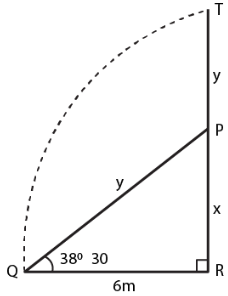In right triangle PQR

tan θ = PR/QR

Substituting the values

tan 380 30’ = x/6

x/6 = 0.7954

By cross multiplication

x = 0.7954 × 6 = 4.7724

We know that

sin θ = PR/PQ

Substituting the values

sin 380 30’ = x/y

So we get

0.6225 = 4.7724/y

y = 4.7724/0.6225 = 7.6665

Here

Height of the tree = 4.7724 + 7.6665 = 12.4389 = 12.44m

Height of the tree at which it is broken = 4.77 m

13. An observer 1.5 m tall is 20.5 metres away from a tower 22 metres high. Determine the angle of elevation of the top of the tower from the eye of the observer.

Solution:

In the figure,

AB is the tower and CD is an observer

θ is the angle of observation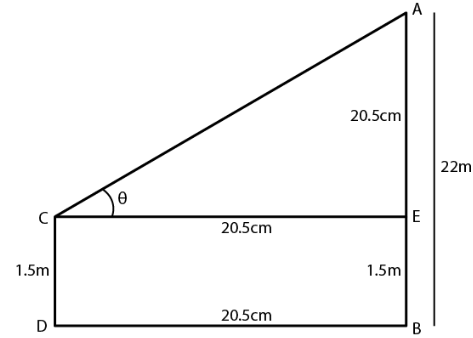It is given that

AB = 22m

CD = 1.5 m

Distance BD = 20.5 m

From the point C construct CE parallel tp DB

AE = 22 – 1.5 = 20.5 m

CE = DB = 20.5 m

tan θ = AE/CE

Substituting the values

tan θ = 20.5/20.5 = 1

tan 450 = 1

θ = 450

14. (i) In the adjoining figure, the angle of elevation from a point P of the top of a tower QR, 50 m high is 600 and that of the tower PT from a point Q is 300. Find the height of the tower PT, correct to the nearest metre.

(ii) From a boat 300 metres away from a vertical cliff, the angles of elevation of the top and the foot of a vertical concrete pillar at the edge of the cliff are 550 40’ and 540 20’ respectively. Find the height of the pillar correct to the nearest metre.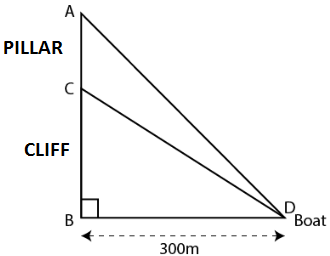Solution:

Consider CB as the cliff and AC as the pillar

D as the boat which is 300 m away from the foot of the cliff BD = 300 m

Angle of elevation of the top and foot of the pillar are 550 40’ and 540 20’

Take CB = x and AC = y

In a right triangle CBD

tan θ = CB/BD

Substituting the values

tan 540 20’ = x/300

So we get

1.3933 = x/300

By cross multiplication

x = 300 × 1.3933

x = 417.99 m

In a right triangle ABD

tan θ = AB/BD

Substituting the values

(x + y)/ 300 = 1.4641

By cross multiplication

x + y = 1.4641 × 300 = 439.23

Substituting the value of x

y = 439.23 – 417.99 = 21.24 m = 21 m

Hence, the height of pillar is 21 m.

15. From a point P on the ground, the angle of elevation of the top of a 10 m tall building and a helicopter hovering over the top of the building are 300 and 600 respectively. Find the height of the helicopter above the ground.

Solution:

Consider AB as the building and H as the helicopter hovering over it

P is a point on the ground

Angle of elevation of the top of building and helicopter are 300 and 600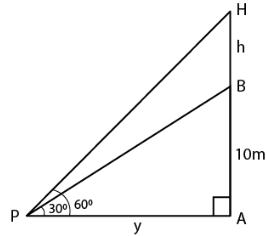We know that

Height of the building AB = 10 m

Take PA = x m and BH = h m

In right triangle ABP

tan θ = P/B

Substituting the values

tan 300 = AB/PA = 10/x

So we get

1/√3 = 10/x

x = 10√3 m

In right triangle APH

tan 600 = AH/PA

tan 600 = (10 + h)/ x

So we get

√3 = (10 + h)/ 10√3

By further calculation

10√3 × √3 = 10 + h

30 = 10 + h

h = 30 – 10 = 20

Height of the helicopter from the ground = 10 + 20 = 30 m

16. An aeroplane when flying at a heigt of 3125 m from the ground passes vertically below another plane at an instant when the angles of elevation of the two planes from the same point on the ground are 300 and 600 respectively. Find the distance between the two planes at the instant.

Solution:

Consider the distance between two planes = h m

It is given that

AD = 3125 m, ∠ACB = 600 and ∠ACD = 300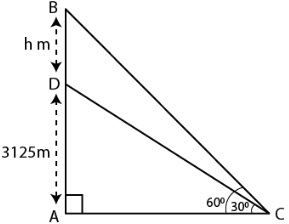In triangle ACD

Substituting the values

1/√3 = 3125/AC

AC = 3125√3 ……. (1)

In triangle ABC

tan 600 = AB/AC

Substituting the values

√3 = (AD + DB)/ AC

So we get

√3 = (3125 + h)/ AC

AC = (3125 + h)/ √3 ….. (2)

Using both the equations

(3125 + h)/ √3 = 3125√3

By further calculation

h = (3125√3 × √3) – 3125

h = 3125 × 3 – 3125

h = 9375 – 3125

h = 6250 m

Therefore, the distance between two planes at the instant is 6250 m.

17. A person standing on the bank of a river observes that the angle subtended by a tree on the opposite bank is 600; when he retires 20 m from the bank, he finds the angle to be 300. Find the height of the tree and the breadth of the river.

Solution:

Consider TR as the tree and PR as the width of the river.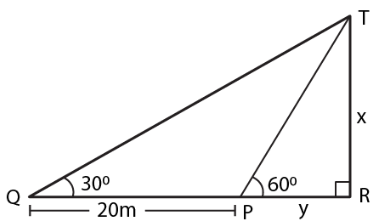Take TR = X and PR = y

In right triangle TPR

tan θ = TR/PR

Substituting the values

tan 600 = x/y

So we get

√3 = x/y

x = y √3…… (1)

In right triangle TQR

tan 300 = TR/QR

tan 300 = x/(y + 20)

We get

1/√3 = x/(y + 20)

x = (y + 20)/ √3 ….. (2)

Using both the equations

y√3 = (y + 20)/ √3

So we get

3y = y + 20

3y – y = 20

2y = 20

y = 10

Now substituting the value of y in equation (1)

x = 10 × √3 = 10 (1.732) = 17.32

Hence, the height of the tree is 17.32 m and the width of the river is 10 m.

18. The shadow of a vertical tower on a level ground increases by 10 m when the altitude of the sun changes from 450 to 300. Find the height of the tower, correct to two decimal places.

Solution:

In the figure

AB is the tower

BD and BC are the shadow of the tower in two situations

Consider BD = x m and AB = h m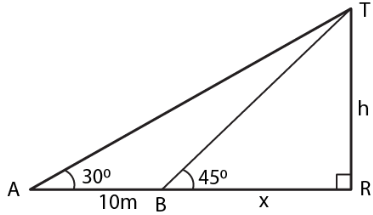In triangle ABD

tan 450 = h/x

So we get

1 = h/x

h = x ….. (1)

In triangle ABC

tan 300 = h/(x + 10)

So we get

1/√3 = h/(x + 10)

Using equation (1)

h√3 = h + 10

h (√3 – 1) = 10

We know that

h = 10/(√3 – 1)

It can be written as

h = [10 (√3 + 1)]/ [(√3 – 1) (√3 + 1)]

By further calculation

h = (10√3 + 1)/ 2

So we get

h = 5 (1.73 + 1)

h = 5 × 2.73

h = 13.65 m

Therefore, the height of the tower is 13.65 m.

19. From the top of a hill, the angles of depression of two consecutive kilometer stones, due east are found to be 300 and 450 respectively. Find the distance of two stones from the foot of the hill.

Solution:

Consider A and B as the position of two consecutive kilometre stones

Here AB = 1 km = 1000 m

Take Distance BC = x m

Distance AC = (1000 + x) m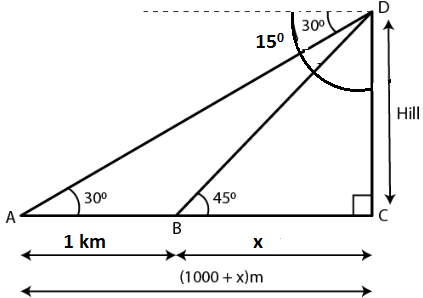In right angled triangle BCD

CD/BC = tan 450

So we get

CD/BC = 1

CD = BC = x

In right angled triangle ACD

DC/AC = tan 300

x/ (x + 1000) = 1/√3

By cross multiplication

√3x = x + 1000

(√3 – 1)x = 1000

x = 1000/ (√3 – 1)

We can write it as

x = [1000/(√3 – 1) × (√3 + 1)/ (√3 + 1)]

x = [1000 (√3 + 1)/ (3 – 1)]

x = [1000 (√3 + 1)/ 2]

x = 500 (1.73 + 1)

So we get

x = 500 × 2.73

x = 1365 m

Here the distance of first stone from the foot of hill = 1365 m

Distance of the second stone from the foot of hill = 1000 + 1365 = 2365 m

20. A man observes the angles of elevation of the top of a building to be 300. He walks towards it in a horizontal line through its base. On covering 60 m the angle of elevation changes to 600. Find the height of the building correct to the nearest decimal place.

Solution:

It is given that

AB is a building

CD = 60 m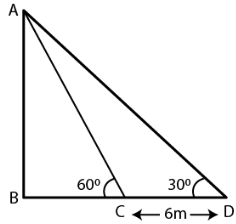In triangle ABC

tan 600 = AB/BC

√3 = AB/BC

So we get

BC = AB/√3 ….. (1)

In triangle ABD

tan 300 = AB/BD

1/√3 = AB/ (BC + 60)

By cross multiplication

BC + 60 = √3 AB

BC = √3 AB – 60

Using both the equations we get

AB/√3 = √3 AB – 60

By further calculation

AB = 3AB – 60√3

3AB – AB = 60 × 1.732

So we get

AB = (60 × 1.732)/ 2

AB = 51.96 m

21. At a point on level ground, the angle of elevation of a vertical lower is found to be such that its tangent is 5/12. On walking 192 m towards the tower, the tangent of the angle is found to be ¾. Find the height of the tower.

Solution:

Consider TR as the tower and P as the point on the ground such that tan θ = 5/12

tan α = ¾

PQ = 192 m

Take TR = x and QR = y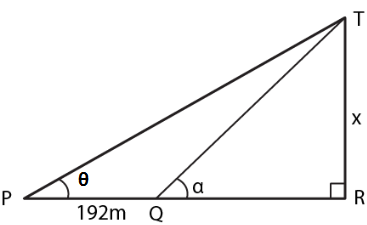In right triangle TQR

tan α = TR/QR = x\y

So we get

3/4 = x/y

y = 4/3 x …..(1)

In right triangle TPR

tan θ = TR/PR

Substituting the values

5/12 = x/ (y + 192)

x = (y + 192) 5/12 …… (2)

Using both the equations

x = (4/3 x + 192) 5/12

So we get

x = 5/9x + 80

x – 5/9 x = 80

4/9 x = 80

By further calculation

x = (80 × 9)/ 4 = 180

Hence, the height of the tower is 180 m.

22. In the figure, not drawn to scale, TF is a tower. The elevation of T from A is x0 where tan x = 2/5 and AF = 200 m. The elevation of T from B, where AB = 80 m, is y0. Calculate:

(i) the height of the tower TF.

(ii) the angle y, correct to the nearest degree.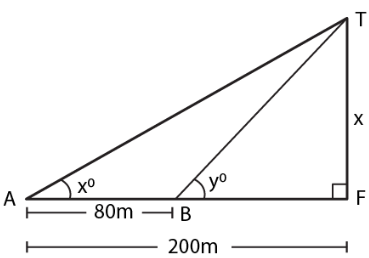Solution:

Consider the height of the tower TF = x

It is given that

tan x = 2/5, AF = 200 m, AB = 80 m

(i) In right triangle ATF

tan x0 = TF/AF

Substituting the values

2/5 = x/200

So we get

x = (2 × 200)/ 5

x = 400/5

x = 80 m

Hence, the height of tower is 80 m.

(ii) In right triangle TBF

tan y = TF/BF

Substituting the values

tan y = 80/ (200 – 80)

tan y = 80/120

tan y = 2/3 = 0.6667

So we get

y = 330 41’ = 340

23. From the top of a church spire 96 m high, the angles of depression of two vehicles on a road, at the same level as the base of the spire and on the same side of it are x0 and y0, where tan x0 = ¼ and tan y0 = 1/7. Calculate the distance between the vehicles.

Solution:

Consider CH as the height of the church

A and B are two vehicles which make an angle of depression x0 and y0 from C

Take AH = x and BH = yIn a right triangle CBH

tan x0 = CH/AH = 96/y

Substituting the values

1/4 = 96/y

So we get

y = 96 × 4 = 384 m

In right triangle CAH

tan y0 = CH/AH = 96/x

Substituting the values

1/7 = 96/x

So we get

x = 96 × 7 = 672 m

Here

AB = x – y

AB = 672 – 384

AB = 288 m

24. In the adjoining figure, not drawn to the scale, AB is a tower and two objects C and D are located on the ground, on the same side of AB. When observed from the top A of the tower, their angles of depression are 450 and 600. Find the distance between the two objects. If the height of the tower is 300. Give your answer to the nearest metre.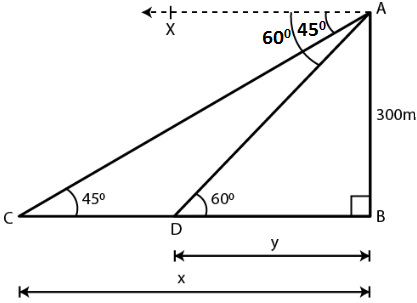Solution:

Consider CB = x and DB = y

AB = 300 m

In right triangle ACD

tan θ = AB/CB

Substituting the values

tan 450 = 300/x

1 = 300/x

So we get

x = 300 m

tan θ = AB/DB

Substituting the values

tan 600 = 300/y

√3 = 300/y

By further calculation

y = 300/√3

Multiply and divide by √3

y = 300/√3 × √3/√3 = 300√3/3

So we get

y = 100 × 1.732 = 173.2 m

Here

CD = x – y = 300 – 173.2 = 126.8 = 127 m

Hence, the distance between two objects is 127 m.

25. The horizontal distance between two towers is 140 m. The angle of elevation of the top of the first tower when seen from the top of the second tower is 300. If the height of the second tower is 60 m, find the height of the first tower.

Solution:

Consider the height of the first tower TR = x

It is given that

Height of the second tower PQ = 60 m

Distance between the two towers QR = 140 m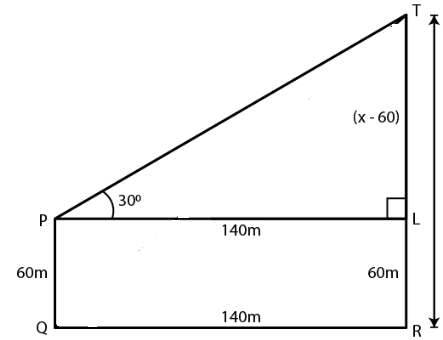Construct PL parallel to QR

LR = PQ = 60 m

PL = QR = 140 m

So we get

TL = (x – 60) m

In right triangle TPL

tan θ = TL/PL

Substituting the values

tan 300 = (x – 60)/ 140

So we get

1/√3 = (x – 60)/ 140

By further calculation

x – 60 = 140/√3

Multiply and divide by √3

x – 60 = 140/√3 × √3/√3 = 140√3/3

We get

x = 140√3/3 + 60

x = (140 × 1.732)/ 3 + 60

x = 80.83 + 60

x = 140.83

Hence, the height of first tower is 140.83 m.

26. As observed from the top of a 80 m tall light house, the angles of depression of two ships on the same side of the light house in horizontal line with its base are 300 and 400 respectively. Find the distance between the two ships. Give your answer correct to the nearest metre.

Solution:

Consider AB as the light house and C and D as the two ships.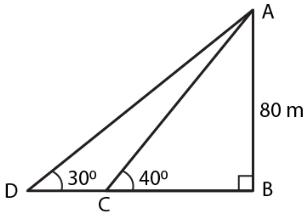tan 300 = AB/BD

Substituting the values

1/√3 = 80/BD

So we get

BD = 80√3…… (1)

In triangle ACB

tan 400 = AB/BC

Substituting the values

0.84 = 80/BC

So we get

BC = 80/0.84 = 95.25

Using equation (1)

BD = 80 √3 = 80 × 1.73 = 138.4

Here we get

DC = BD – BC

DC = 138.4 – 95.25 = 43.15

Therefore, the distance between the two ships is 43.15 m.

27. The angle of elevation of a pillar from a point A on the ground is 450 and from a point B diametrically opposite to A and on the other side of the pillar is 600. Find the height of the pillar, given that the distance between A and B is 15 m.

Solution:

Consider CD as the pillar of x m

Angles of elevation of points A and B are 450 and 600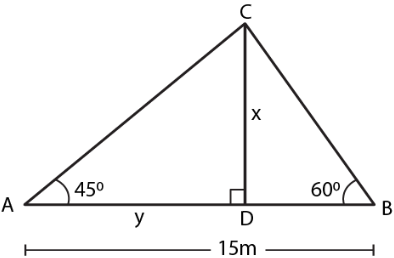It is given that

AB = 15 m

DB = 15 – y

Substituting the values

tan 450 = x/y

So we get

1 = x/y

x = y …… (1)

In triangle CDB

tan 600 = x/(15 – y)

Substituting the values

√3 = x/(15 – y)

So we get

x = √3 (15 – y) ….. (2)

Using both the equations

x = √3 (15 – x)

x = 15√3 – √3x

So we get

x + √3x = 15√3

x (1 + √3) = 15√3

x = 15√3/ (1 + √3)

We can write it as

x = (15 × 1.732)/ (1 + 1.732)

x = 25.98/2.732

x = 9.51

Hence, the height of the pillar is 9.51 m.

28. From two points A and B on the same side of a building, the angles of elevation of the top of the building are 300 and 600 respectively. If the height of the building is 10 m, find the distance between A and B correct to two decimal places.

Solution: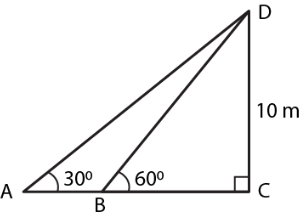In triangle DBC

tan 600 = 10/BC

Substituting the values

√3 = 10/BC

BC = 10/√3

In triangle DBC

tan 300 = 10/ (BC + AB)

Substituting the values

1/√3 = 10/[10/√3 + AB]

By further calculation

1/√3 [10/√3 + AB] = 10

So we get

AB = 10√3 – 10/√3

Taking LCM

AB = (30 – 10)/ √3

AB = 20/√3

AB = 20√3/3

So we get

AB = (20 × 1.732)/ 3

AB = 20 × 0.577

AB = 11.540 m

Hence, the distance between A and B is 11.54 m.

29. (i) The angles of depression of two ships A and B as observed from the top of a light house 60 m high are 600 and 450 respectively. If the two ships are on the opposite sides of the light house, find the distance between the two ships. Give your answer correct to the nearest whole number.

(ii) An aeroplane at an altitude of 250 m observes the angle of depression of two boats on the opposite banks of a river to be 450 and 600 respectively. Find the width of the river. Write the answer correct to the nearest whole number.

Solution:

(i) Consider AD as the height of the light house CD = 60 m

Take AD = x m and BD = y m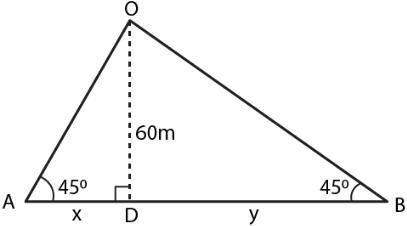In triangle ACD

Substituting the values

√3 = 60/x

So we get

x = 60/√3

Multiply and divide by √3

x = 60/√3 × √3/√3 = 60√3/3

x = 20 × 1.732 = 34.64 m

In triangle BCD

tan 450 = CD/BD

Substituting the values

1 = 60/y

y = 60 m

Here the distance between two ships = x + y

= 34.64 + 60

= 94.64 m

= 95 m

(ii) In triangle OMA

tan 450 = OM/AM

Substituting the values

1 = 250/x

So we get

x = 250 m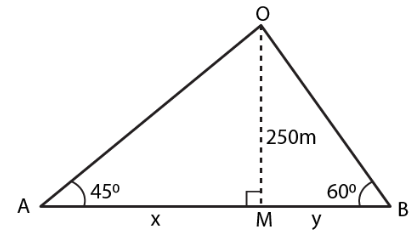In triangle OMB

tan 600 = 250/y

Substituting the values

√3 = 250/y

So we get

y = 250/√3 = 250/1.73

y = 144.34

Here

Width of the river = x + y

Substituting the values

= 250 + 144.34

= 394.34 m

30. From a tower 126 m high, the angles of depression of two rocks which are in a horizontal line through the base of the tower are 160 and 120 20’. Find the distance between the rocks if they are on

(i) the same side of the tower

(ii) the opposite sides of the tower.

Solution:

Consider CD as the tower of height = 126 m

A and B are the two rocks on the same line

Angles of depression are 160 and 120 20’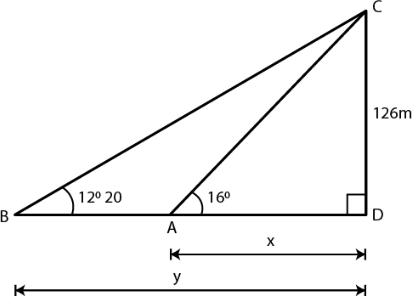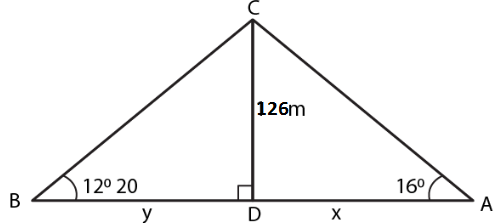Substituting the values

tan 160 = 126/x

0.2867 = 126/x

So we get

x = 126/0.2867

x = 439.48

In right triangle CBD

tan 120 20’ = 126/y

So we get

0.2186 = 126/y

y = 126/0.2186 = 576.40

(i) In the first case

On the same side of the tower

AB = y – x

Substituting the values

AB = 576.40 – 439.48

AB = 136.92 m

(ii) In the second case

On the opposite side of the tower

AB = y + x

Substituting the values

AB = 576.40 + 439.48

AB = 1015.88m

31. A man 1.8 m high stands at a distance of 3.6 m from a lamp post and casts a shadow of 5.4 m on the ground. Find the height of the lamp post.

Solution:

Consider

AB as the lamp post

CD is the height of man

BD is the distance of man from the foot of the lamp

FD is the shadow of man

Construct CE parallel to DB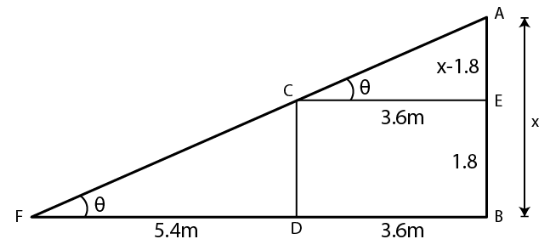Take AB = x and CD = 1.8 m

EB = CD = 1.8 m

AE = x – 1.8

In right triangle ACE

tan θ = AE/CE

Substituting the values

tan θ = (x – 1.8)/ 3.6 …… (1)

In right triangle CFD

tan θ = CD/FD

Substituting the values

tan θ = 1.8/5.4 = 1/3 ….. (2)

Using both the equations

(x – 1.8)/ 3.6 = 1/3

So we get

3x – 5.4 = 3.6

3x = 3.6 + 5.4 = 9.0

By division

x = 9/3 = 3.0

Hence, the height of lamp post is 3 m.

32. The angles of depression of the top and the bottom of an 8 m tall building from the top of a multi-storeyed building are 300 and 450 respectively. Find the height of tire multi-storeyed building and the distance between the two buildings, correct to two decimal places.

Solution:

Consider AB as the height and CD as the building

The angles of depression from A to C and D are 300 and 450

∠ACE = 300 and ∠ADB = 450

CD = 8 m

Take AB = h and BD = x

From the point C

Construct CE parallel to DB

CE = DB = x

EB = CD = 8 m

AR = AB – EB = h – 8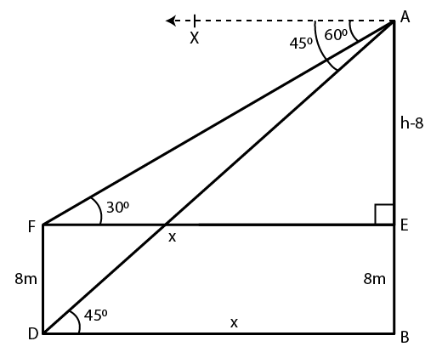tan θ = AB/DB

Substituting the values

tan 450 = h/x

So we get

1 = h/x

x = h

In right triangle ACE

tan 300 = AE/CE

Substituting the values

1/√3 = (h – 8)/ h

By further calculation

h = √3h – 8√3

So we get

√3h – h = 8√3

h (√3 – 1) = 8√3

h = 8√3/(√3 – 1)

Multiply and divide by √3 + 1

h = 8√3/ (√3 – 1) × (√3 + 1)/ (√3 + 1)

h = 8 (3 + √3)/ (3 – 1)

Here

h = 8 (3 + 1.732)/ 2

h = 4 × 4.732

h = 18.928

h = 18.93 m

x = h = 18.93 m

Here

Height of multi-storeyed building = 18.93 m

Distance between the two buildings = 18.93 m

33. A pole of height 5 m is fixed on the top of a tower. The angle of elevation of the top of the pole as observed from a point A on the ground is 600 and the angle of depression of the point A from the top of the tower is 450. Find the height of the tower. (Take √3 = 1.732)

Solution:

Consider QR as the tower

PQ as the pole on it

Angle of elevation from P to a point A is ∠PAR = 600

Angle of depression from Q to A = 450

Here

∠QAR = 450 which is the alternate angle

PQ = 5 m

Take QR = h m

PQ = 5 + h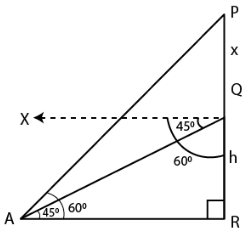In right triangle QAR

tan θ = QR/AR

Substituting the values

tan 450 = h/AR

So we get

1 = h/AR

AR = h

In right triangle PAR

tan 600 = PR/AR

Substituting the values

√3 = (5 + h)/ h

So we get

√3h = 5 + h

h (√3 – 1) = 5

h (1.732 – 1) = 5

By further calculation

0.732 h = 5

h = 5/0.732 = 5000/732

h = 6.83

Hence, the height of the tower is 6.83 m.

34. A vertical pole and a vertical tower are on the same level ground. From the top of the pole the angle of elevation of the top of the tower is 600 and the angle of depression of the foot of the tower is 300. Find the height of the tower if the height of the pole is 20 m.

Solution:

Consider TR as the tower

PL as the pole on the same level

Ground PL = 20 m

From the point P construct PQ parallel to LR

∠TPQ = 600 and ∠QPR = 300

Here

∠PRL = ∠QPR = 300 which are the alternate angles

Take LR = x and TR = h

TQ = TR – QR = (h – 20) m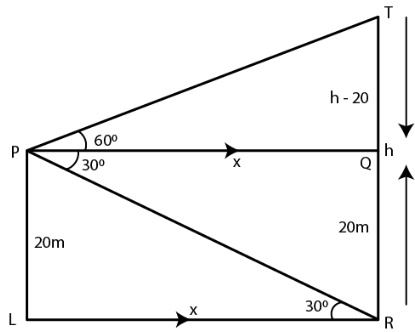In right triangle PRL

tan θ = PL/LR

Substituting the values

tan 300 = 20/x

So we get

1/√3 = 20/x

x = 20√3 m

In right triangle PQT

tan 600 = TQ/PQ

Substituting the values

√3 = (h – 20)/ x

√3 = (h – 20)/ 20√3

By cross multiplication

20√3 × √3 = h – 20

20 × 3 = h – 20

h = 60 + 20 = 80 m

Hence, the height of the tower is 80 m.

35. From the top of a building 20 m high, the angle of elevation of the top of a monument is 450 and the angle of depression of its foot is 150. Find the height of the monument.

Solution:

Consider AB as the building where AB = 20 m

CD as the monument where CD = x m

Take the distance between the building and the monument as y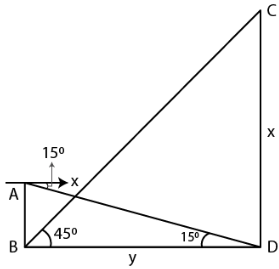In right triangle BCD

tan θ = CD/BD

Substituting the values

tan 450 = x/y

1 = x/y

x = y …… (1)

In right triangle ABD

tan 150 = AB/BD = 20/x

Substituting the values

0.2679 = 20/x

So we get

x = 20/0.2679 = 74.65 m

Hence, the height of the monument is 74.65 m.

36. The angle of elevation of the top of an unfinished tower at a point distant 120 m from its base is 450. How much higher must the tower be raised so that its angle of elevation at the same point may be 600?

Solution:

Consider AB as the unfinished tower where AB = 120 m

Angle of elevation = 450

Take x be higher raised so that the angle of elevation becomes 600

BC = y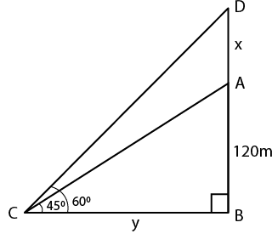In right triangle ABC

tan θ = AB/CB

Substituting the values

tan 450 = AB/CB = 120/y

So we get

1 = 120/y

y = 120 m

In right triangle DBC

tan 600 = DB/CB

Substituting the values

√3 = (120 + x)/ 120

120√3 = 120 + x

x = 120√3 – 120

x = 120 (√3 – 1)

So we get

x = 120 (1.732 – 1)

x = 120 (0.732)

x = 87.84 m

Hence, the tower should be raised at 87.84 m.

37. In the adjoining figure, the shadow of a vertical tower on the level ground increases by 10 m, when the altitude of the sun changes from 450 to 300. Find the height of the tower and give your answer, correct to 1/10 of a metre.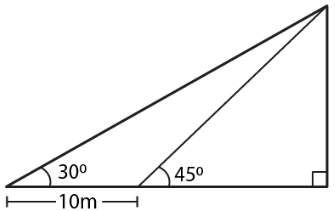Solution:

Consider TR as the tower where TR = h

BR = x

AB = 10 m

Angles of elevation from the top of the tower at A and B are 300 and 450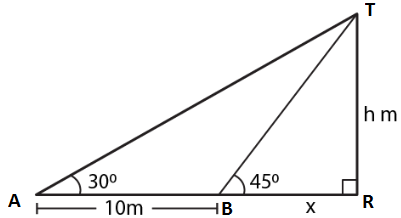In right triangle TAR

tan θ = TR/AR

Substituting the values

tan 300 = h/ (10 + x)

So we get

1/√3 = h/ (10 + x)

h = (10 + x)/ √3 …. (1)

In triangle TBR

tan 450 = TR/BR = h/x

So we get

1 = h/x

x = h ….. (2)

Using both the equations

h = (10 + h)/ √3

√3h = 10 + h

By further calculation

√3h – h = 10

(1.732 – 1) h = 10

0.732 h = 10

h = 10/0.732 = 13.66

Hence, the height of the tower is 13.7 m.

38. An aircraft is flying at a constant height with a speed of 360 km/h. From a point on the ground, the angle of elevation of the aircraft at an instant was observed to be 450. After 20 seconds, the angle of elevation was observed to be 300. Determine the height at which the aircraft flying. (use √3 = 1.732)

Solution:

It is given that

Speed of aircraft = 360 km/h

Distance covered by the aircraft in 20 seconds = (360 × 20)/ (60 × 60) = 2 km

Consider E as the fixed point on the ground

CD as the position of AB in height of aircraft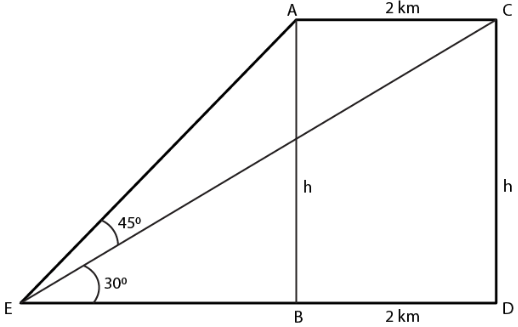Take AB = CD = h km

In right triangle ARB

tan θ = AB/ EB

Substituting the values

tan 450 = h/EB

1 = h/EB

EB = h

Here

ED = EB + BD = h + 2 km

In right triangle CED

tan 300 = CD/ED

Substituting the values

1/√3 = h/ (h + 2)

√3h = h + 2

1.732h – h = 2

0.732h = 2

We know that 2km = 2000 m

h = 2000/0.732

h = (2000 × 1000)/ 732 = 2732 m

Chapter Test

1. The angle of elevation of the top of a tower from a point A (on the ground) is 300. On walking 50 m towards the tower, the angle of elevation is found to be 600. Calculate

(i) the height of the tower (correct to one decimal place).

(ii) the distance of the tower from A.

Solution:

Consider TR as the tower and A as the point on the ground

Angle of elevation of the top of tower = 300

AB = 50 m

Angle of elevation from B = 600

Take TR = h and AR = x

BR = x – 50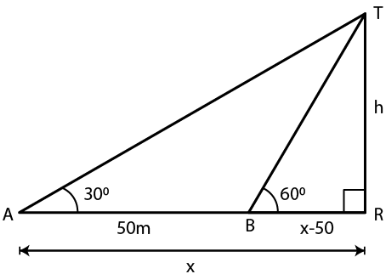In right triangle ATR

tan θ = TR/AR

Substituting the values

tan 300 = h/x

So we get

1/√3 = h/x

x = √3h ……. (1)

In right triangle BTR

tan θ = TR/BR

Substituting the values

tan 600 = h/ (x – 50)

So we get

√3 = h/ (x – 50)

h = √3 (x – 50) …… (2)

Using both the equations

h = √3 (√3h – 50)

By further calculation

h = 3h – 50√3

2h = 50√3

h = 25 √3

So we get

h = 25 × 1.732 = 43.3

Now substituting the values of h in equation (1)

x = √3 × 25√3

x = 25 × 3

x = 75

Here

Height of the tower = 43.3 m

Distance of A from the foot of the tower = 75 m

2. An aeroplane 3000 m high passes vertically above another aeroplane at an instant when the angles of elevation of the two aeroplanes from the same point on the ground are 600 and 450 respectively. Find the vertical distance between the two planes.

Solution:

Consider A and B as the two aeroplanes

P is a point on the ground such that 600 and 450 are the angles of elevations from A and B

AC = 3000 m

Take AC = 3000 m

BC = 3000 – x

PC = y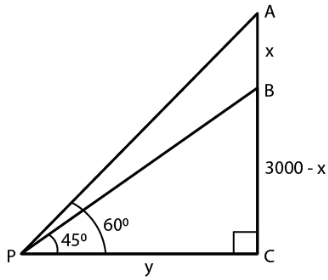In right triangle APC

tan θ = AC/PC

Substituting the values

tan 600 = 3000/y

So we get

√3 = 3000/y

y = 3000/√3 ….. (1)

In right triangle BPC

tan θ = BC/PC

Substituting the values

tan 450 = (3000 – x)/ y

So we get

1 = (3000 – x)/ y

y = 3000 – x

Using equation (1)

3000/√3 = 3000 – x

By further calculation

x = 3000 – 3000/√3

Multiply and divide by √3

x = 3000 – (3000 × √3)/ (√3× √3)

So we get

x = 3000 – 1000 (1.732)

x = 3000 – 1732

x = 1268

Hence, the distance between the two planes is 1268 m.

3. A 7 m long flagstaff is fixed on the top of a tower. From a point on the ground, the angles of elevation of the top and bottom of the flagstaff are 450 and 360 respectively. Find the height of the tower correct to one place of decimal.

Solution:

Consider TR as the tower and PT as the flag on it

PT = 7 m

Take TR = h and AR = x

Angles of elevation from P and T are 450 and 360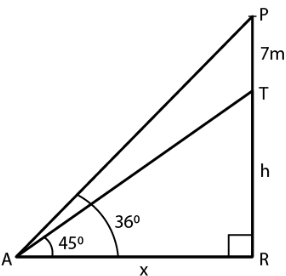In right triangle PAR

tan θ = PR/AR

Substituting the values

tan 450 = (7 + h)/ x

So we get

1 = (7 + h)/ x

x = 7 + h …. (1)

In right triangle TAR

tan θ = TR/AR

Substituting the values

tan 360 = h/x

So we get

0.7265 = h/x

h = x (0.7265) …… (2)

Using both the equations

h = (7 + h) (0.7265)

By further calculation

h = 7 × 0.7265 + 0.7265h

h – 0.7265h = 7 × 0.7265

So we get

0.2735h = 7 × 0.7265

By division

h = (7 × 0.7265)/ 0.2735

We can write it as

h = (7 × 7265)/ 2735

h = 18.59 = 18.6 m

Hence, the height of the tower is 18.6 m.

4. A boy 1.6 m tall is 20 m away from a tower and observes that the angle of elevation of the top of the tower is 600. Find the height of the tower.

Solution:

Consider AB as the boy and TR as the tower

AB = 1.6 m

Take TR = h

From the point A construct AE parallel to BR

ER = AB = 1.6 m

TE = h – 1.6

AE = BR = 20 m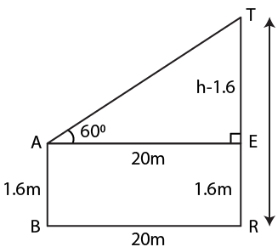In right triangle TAE

tan θ = TE/AE

Substituting the values

tan 600 = (h – 1.6)/ 20

So we get

√3 = (h – 1.6)/ 20

h – 1.6 = 20√3

h = 20√3 + 1.6

h = 20 (1.732) + 1.6

By further calculation

h = 34.640 + 1.6

h = 36.24

Hence, the height of the tower is 36.24 m.

5. A boy 1.54 m tall can just see the sun over a wall 3.64 m high which is 2.1 m away from him. Find the angle of elevation of the sun.

Solution:

Consider AB as the boy and CD as the wall which is at a distance of 2.1 m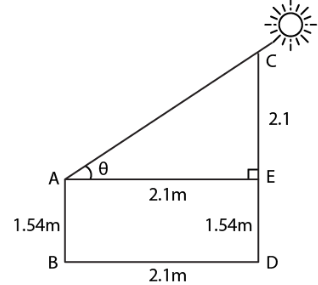AB = 1.54 m

CD = 3.64 m

BD = 2.1 m

Construct AE parallel to BD

ED = 1.54 m

CE = 3.64 – 1.54 = 2.1 m

AE = BD = 2.1 m

In right triangle CAE

tan θ = CE/AE

So we get

tan θ = 2.1/2.1

tan θ = 21/21

tan θ = 1

We know that

tan 450 = 1

We get

θ = 450

Hence, the angle of elevation of the sun is 450.

6. In the adjoining figure, the angle of elevation of the top P of a vertical tower from a point X is 600; at a point Y, 40 m vertically above X, the angle of elevation is 450. Find

(i) the height of the tower PQ

(ii) the distance XQ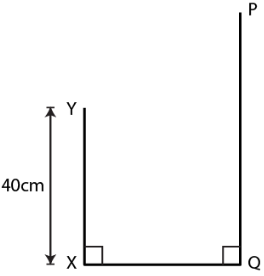Solution:

Consider PQ as the tower = h

XQ = YR = y

XY = 40 m

PR = h – 40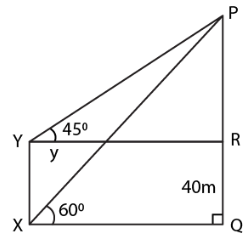In right triangle PXQ

tan θ = PQ/XQ

Substituting the values

tan 600 = h/y

So we get

√3 = h/y

y = h/√3 ….. (1)

In right triangle PYR

tan θ = PR/YR

Substituting the values

tan 450 = (h – 40)/ y

So we get

1 = (h – 40)/ y

y = h – 40 …… (2)

Using both the equations

h – 40 = h/√3

By further calculation

√3h – 40√3 = h

√3h – h = 40√3

So we get

(1.732 – 1)h = 40 (1.732)

732h = 69.280

By division

h = 69.280/0.732 = 69280/732 = 94.64

Here

Height of the tower = 94.64 m = 95 m

Distance XQ = h – y = 95 – 40 = 55 m

7. An aeroplane is flying horizontally 1 km above the ground is observed at an elevation of 600. After 10 seconds, its elevation is observed to be 300. Find the speed of the aeroplane in km/hr.

Solution:

Consider A and D as the two positions of the aeroplane

AB is the height and P is the point

AB = 1 km

Take AD = x and PB = y

Angles of elevation from A and D at the point P are 600 and 300

Construct DC perpendicular to PB

DC = AB = 1 km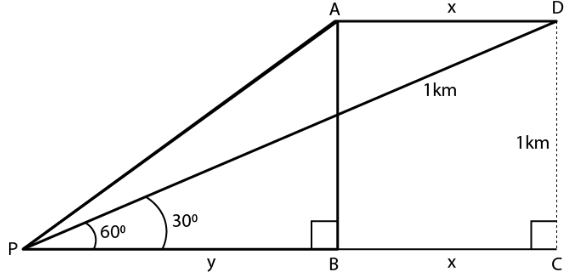In right triangle APB

tan θ = AB/PB

Substituting the values

tan 600 = 1/y

So we get

√3 = 1/y

y = 1/√3 ….. (1)

In right triangle DPC

tan θ = DC/PC

Substituting the values

tan 300 = 1/ (x + y)

So we get

1/√3 = 1/ (x + y)

x + y = √3 ….. (2)

Using both the equations

x + 1/√3 = √3

By further calculation

x = √3 – 1/√3

x = (3 – 1)/ √3

x = 2/√3

Multiply and divide by √3

x = (2 × √3)/ (√3 × √3)

So we get

x = (2 × 1.732)/ 3

x = 3.464/3 km

This distance is covered in 10 seconds

Speed of aeroplane (in km/hr) = 3.464/3 × (60 × 60)/ 10

By further calculation

= 3464/ (3 × 1000) × 3600/10

So we get

= (3646 × 36)/ 300

= (3464 × 12)/ 100

= 41568/ 100

= 415.68 km/hr

8. A man on the deck of a ship is 16 m above the water level. He observes that the angle of elevation of the top of a cliff is 450 and the angle of depression of the base is 300. Calculate the distance of the cliff from the ship and the height of the cliff.

Solution:

Consider A as the man on the deck of a ship B and CE is the cliff

AB = 16 m

Angle of elevation from the top of the cliff = 450

Angle of depression at the base of the cliff = 300

Take CE = h, AD = x

CD = h – 16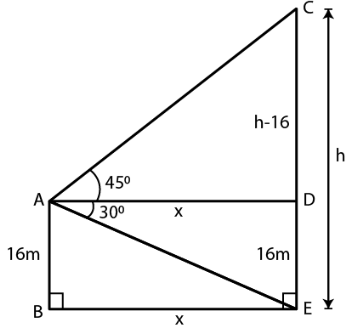Substituting the values

tan 450 = (h – 16)/ x

So we get

1 = (h – 16)/ x

x = h – 16 ……. (1)

Substituting the values

tan 300 = 16/x

So we get

1/√3 = 16/x

x = 16√3 …… (2)

Using both the equations

h – 16 = 16 √3

h = 16√3 + 16

Taking out the common terms

h = 16 (1.732 + 1)

h = 16 (2.732)

h = 43.712 = 43.71 m

Substituting the value in equation (1)

x = h – 16

x = 43.71 – 16

x = 27.71

Here

Distance of cliff = 27.71 m

Height of cliff = 43.71 m

9. There is a small island in between a river 100 metres wide. A tall tree stands on the island. P and Q are points directly opposite to each other on the two banks and in the line with the tree. If the angles of elevation of the top of the tree from P and Q are 300 and 450 respectively, find the height of the tree.

Solution:

Width of the river PQ = 100 m

B is the island and AB is the tree on it

Angles of elevation from A to P and Q are 300 and 450

Consider AB = h

PB = x

BQ = 100 – x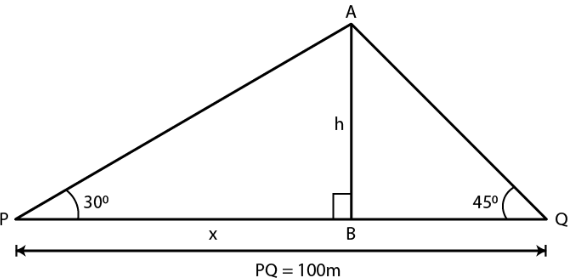In right triangle APB

tan θ = AB/PB

Substituting the values

tan 300 = h/x

So we get

1/√3 = h/x

x = √3h ….. (1)

In right triangle ABQ

tan θ = AB/BQ

Substituting the values

tan 450 = h/ (100 – x)

So we get

1 = h/ (100 – x)

h = 100 – x ….. (2)

Using both the equations

h = 100 – √3h

By further calculation

h + √3h = 100

So we get

(1 + 1.732) h = 100

h = 100/ 2.732

Multiply and divide by 1000

h = (100 × 1000)/ 2732

h = 100000/ 2732

h = 36.6

Hence, the height of the tree is 36.6 m.

10. A man standing on the deck of the ship which is 20 m above the sea-level, observes the angle of elevation of a bird as 300 and the angle of depression of its reflection in the sea as 600. Find the height of the bird.

Solution:

Consider P as the man standing on the deck of the ship which is 20 m above the sea level and B is the bird

Angle of elevation of the bird from P = 300 Angle of depression from P to the shadow of the bird in the sea = 600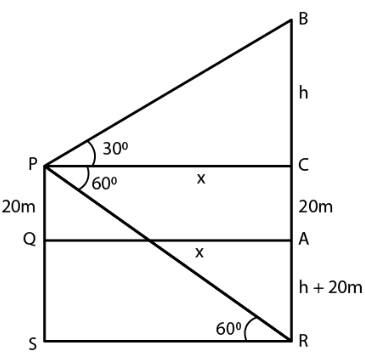Take BC = h

PQ = 20 m = CA

AR = (h + 20) m

CE = h + 20 + 20 = h + 40 m

PC = CA = x

In right triangle PCB

tan 300 = BC/PC

Substituting the values

1/ √3 = h/x

So we get

x = √3h m ……. (1)

In right triangle PCR

tan 600 = CR/PC

Substituting the values

√3 = (h + 40)/ x

Using equation (1)

(h + 40)/ √3h = √3

h + 40 = √3 × √3h = 3h

By further calculation

3h – h = 40

2h = 40

h = 40/2 = 20

From the sea level the height of the bird = 20 + h = 20 + 20 = 40 m